# Efficient Use of The High Motivating Potential of Mathematics to Teach Secondary School Students

London Journal of Research in Humanities and Social Sciences
Volume | Issue | Compilation
Authored by Zhanna Dedovets , M. Rodionov
Classification: FOR CODE- 740201
Keywords: mathematics, motivation, secondary school, students, methodological requirements.
Language: English

Students academic motivation is a key factor in their development and the determination of the effectiveness of their education. Improving motivation is indeed very significant about to courses on secondary school mathematics. This article discusses approaches to the formation of motivation. The authors explore the aesthetic component of motivation motivation in teaching mathematics. ​It also examines methodological requirements for the content of secondary school mathematics, illustrates these by specific examples of mathematical problems.

# Efficient use of the High Motivating Potential of Mathematics to Teach Secondary School Students

## _____________________________________________

1. ### ABSTRACT

Students academic motivation is a key factor in their development and the determination of the effectiveness of their education. Improving motivation is indeed very significant about to courses on secondary school mathematics. This article discusses approaches to the formation of motivation. The authors explore the aesthetic component of motivation motivation in teaching mathematics. It also examines methodological requirements for the content of secondary school mathematics, illustrates these by specific examples of mathematical problems.

Keywords: mathematics, motivation, secondary school, students, methodological requirements.

Author α: Department of Mathematics and Computer Science Teaching, Penza State University, Penza, Russia.

σ: Department of School of Education, The University of West Indies, Trinidad and Tobago.

1. ### INTRODUCTION

It is known that one of the most important factors that have a decisive influence on the learning process and the learning outcomes is motivation. The motivation to learn is the process whereby student cognitive activities acquire a personal sense, creating a stable interest and transforming external tasks into the internal needs of students. The motivation becomes identified with these internal needs, and considered as a ‘stable personality education‘, which synthesizes the complex combination of motives, emotions, needs and interests which encourage the student to undertake specific cognitive activities [6, 19]. This personality is formed during the activity and gives it particular features. Accordingly, motivation is a fundamental condition for the realization and achievement of the purposes of education and one of the key criteria for its effectiveness.

There are two basic approaches to developing the motivation to learn. The first approach focuses on the actions and procedures to ensure the actualization and development of motivation within main psychological and pedagogical laws. The educational strategies which use such practices include problem-based learning (PBL), modular learning and student-centered learning. The second approach requires the analysis of subject activity characteristics to identify the specific features of this discipline which motivate (or demotivate) students to learn mathematics.

Regarding school mathematics, such features include a higher level of abstraction and conceptual thinking, the use of the complex logical structure of many mathematical definitions and theorems, the predominance of logical reasoning over "plausible reasoning," and robust intra- subject communication. They also include the diverse components of task-oriented search activity, such as argument, the construction of geometric configurations transformation formulae and computational operation.

In our observation ‘mass teaching‘ of mathematics practice regarding the formation of motivation is not sufficiently rounded. It relies too heavily on traditional techniques to stimulate learning and cognitive activity in the educational process: the use of task with real content to create the initial problem situation, the organization of gaming activities, acquaintance with historical data and demonstrating interconnections with the humanities for emotional "binding." This approach implicitly assumes that the initial subject motivation must each time be recreated from scratch, ignoring any previously formed personality dispositions of each respective student. In our opinion, this practice is one of the reasons for the findings in a big number of publications, namely that secondary school students have indifferent or negative attitudes towards any subject, in particular mathematics.

Results from a questionnaire produced by the authors for students identified many of the key factors determining negative attitudes towards mathematics. Students described combinations of the following: mathematics not being needed to continue their education and careers, a self- perception of innate insufficient mathematical ability, having a "bad" teacher, an unrealistic expectation of being able to remember a wide variety of algorithms, theorems and formulae, too huge an emphasis on proofs and too difficult terminology and symbolic language. These results suggest that many contemporary students have a weak motivational sphere. which is defective; there are certain semantic barriers, and they are reluctant to perform specific type of mathematical activity.

1. ### “SYSTEM OF MOTIVATIONAL COORDINATES”

There is a need to identify a "system of motivational coordinates." which enables evaluation of the motivational potential of particular mathematical activity. In our view, the best way to do so is to proceed from the distinguishing characteristics of these discrete activities, which have been confirmed during the development of mathematical science. To quote Jacques Hadamard [1, p. 98], "the difference between the student working to solve problems in algebra or geometry and inventive, creative work is a difference in level only, since both have analogous natures." It is well known that the initial impetus for the development of mathe- matical knowledge (like any other) is the need to solve specific practical problems arising during the "functioning" of the individual in the environment. Richard Courant [9, p. 19] points out that: "The way forward in the field of mathematics is via the emergence of needs of a practical character. But once having arisen, it inevitably acquires an internal scale which is beyond the scope of direct usefulness". Solely concentrating on practical needs as the major stimulus for mathematicians is inferior to the pursuit of "aesthetic perfection" and "cognition the world of harmony." The aesthetic factor enables the researcher to choose the optimal way of scientific research [1, 3, 5, 9, 15].

Eric Temple Bell , defined the attributes for the selection of aesthetically pleasing mathematical objects (concepts, facts, theorems, problems, ways of reasoning and so forth). Those attributes, confirmed by other researchers [2, 4, 5], can be formulated as the universal usage and applicability in various areas of mathematics and productivity or promotion on the basis by abstraction and generalization. We can add other criteria described in the literature: a essential contrast between the level of difficulty of the facts and the mathematical instruments which are used; the logical ordering of the harmony of the whole and parts, of the external (the idea of symmetry) and internal (perfection of mathe- matical proof). Elaborating on these characteristics of aesthetically appealing problems in mathematics, Eric Temple Bell cites the task of the construction of regular polygons, solved by Gauss, as an example of a special aesthetic perfection. This problem is known as the result of the organic synthesis of algebra, geometry and number theory, and served as the stimulus for much algebraic research.

All of this allows us to treat “an aesthetic component” of the motivational sphere of student activity activity as:

1. A tendency to generalization and abstraction (W. Sawyer, H. Weyl, J. Newman);
2. An orientation towards visualization of analytical objects using appropriate images                (R. Courant, J. Hadamard, J. Birkhoff);
3. The pursuit of unification or refraction of various mathematical facts and laws across mathematical sectors (У. W. Sawyer, E. Bell, R. Courant);
4. The tendency towards the minimum subjective complexity required to achieve a result           (P. Maupertuis, C. Gauss);
5. The need for logical rationale and evidence as the highest form of expression of aesthetic excellence in mathematics (A. Cauchy, K. Weierstrass).

Note also that in the history of science [3, 8, 9] motivational phenomena were realized in various forms (Peacock’s principle of the permanence of equivalent forms, Maupertuis' principle of the least action, Fermat's method of infinite descent, Jacobi's inversion method and Poncelet’s principles of duality and continuity. At present, while there is not as yet the indisputable evidence base for many mathematical theories, they nonetheless retain a motivational and heuristic value in relation about the content of school mathematics. Hence, they should be used in the educational activities of students.

1. ### METHODOLOGICAL REQUIREMENTS FOR SECONDARY SCHOOL MATHEMATICS CONTENT

Improving the content and structure mathematics content involves, in our opinion, the feasibility of formulating and implementing a set of methodological requirements, which we will illustrate using the algebraic material. We previously noted that these requirements to some extent overlap with each other. Their meaning are the ordering of various directions to enhance the motivational component of school mathematics education. also to place emphasis on informing and helping students discover the most advantageous characteristics of classroom activity.

4.1   The requirement of generalization

The requirement of generalization enables students to develop a holistic view of mathematics. Specific student knowledge about mathematical concepts should not be ad hoc and in isolated, self-contained units. They should be conceptualized as organic elements of a whole. In other words, students must master generalized knowledge, incorporating ideas and methods which cover large classes of mathematical facts, events and particular methods of solutions. It is appropriate to quote a Soviet mathematician A. Khinchin, "don't save time, try to inculcate these ideas in students’ consciousness by all possible means, through a variety of approaches "[20, p.7]."

However, as we have seen, these requirements are not always implemented in school-based practice of teaching mathematics. For example, the intervals method is the best way to solve a single variable inequality. This method is introduced in a general way for continuous functions at the appropriate interval. However, the sets of tasks in the standard textbooks are not oriented to the full use of the possibilities of this method. As presented, it is not possible to demonstrate the general pattern of algebraic inequalities generally and all the features and the "power" of this approach. As a result, during exams, many students and enrollees do not feel the need to apply the method of intervals, although the use of this method makes the solution much more transparent than the reversion to an elementary system of inequalities. In other words, students do not master the method of intervals as a generalized method method of solving problem. This is one of the reasons why students are unable to produce a solution even though they have the capacity to do so. The formulation of the method of intervals used in schools has the following defects: unnecessary detailing of the algorithm ("compulsory" factorization, irrational determination of signs on each of the allocated intervals) and lack of attention to the possibility of isolated solutions.

This situation can be corrected by expanding the number of inequalities that can be solved using the method of intervals. Then this method will, as if tracing a thread, enable students to follow the basic trend of "development" of this way. Each stage of the solution of a new type of inequalities should be accompanied by generalized reflection of the performed procedures.

Problem 1. Solve the given inequality: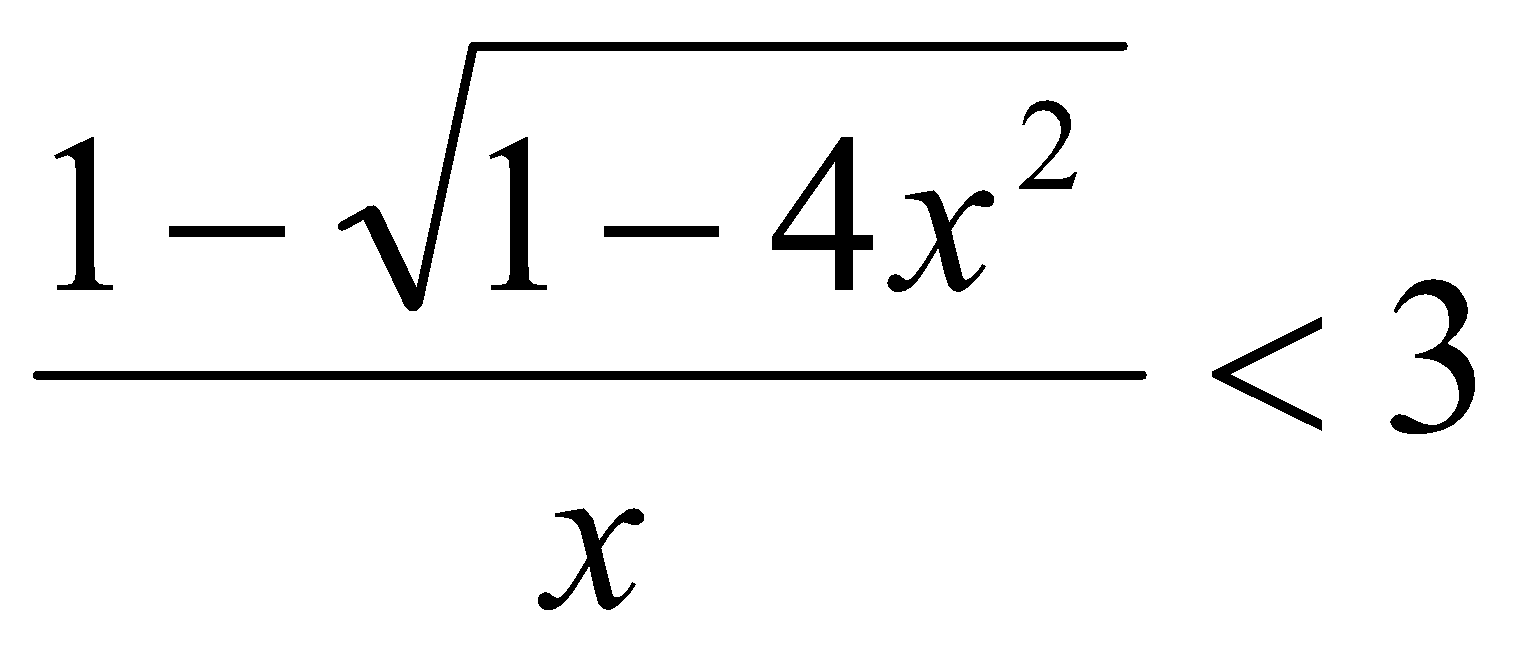To solve this irrational inequality use universal algorithms:

1. Find a basic function. For this transfer the expression from one of the part of the inequality to the other: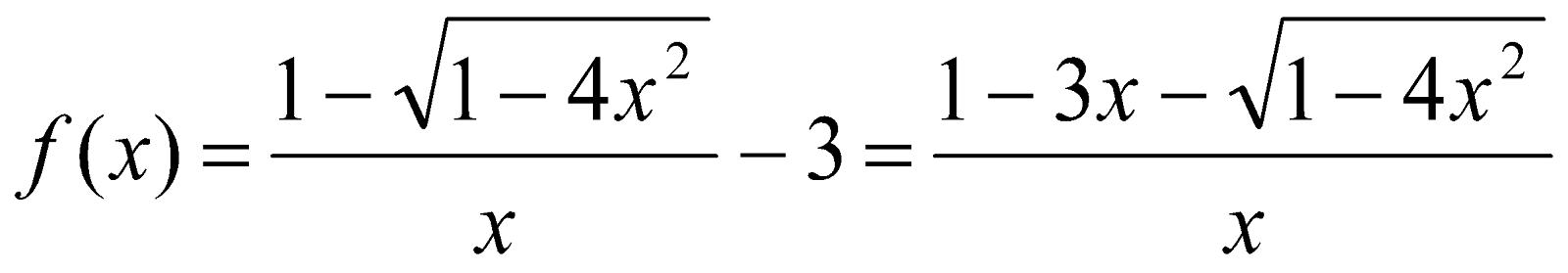1. Find the domain of this function: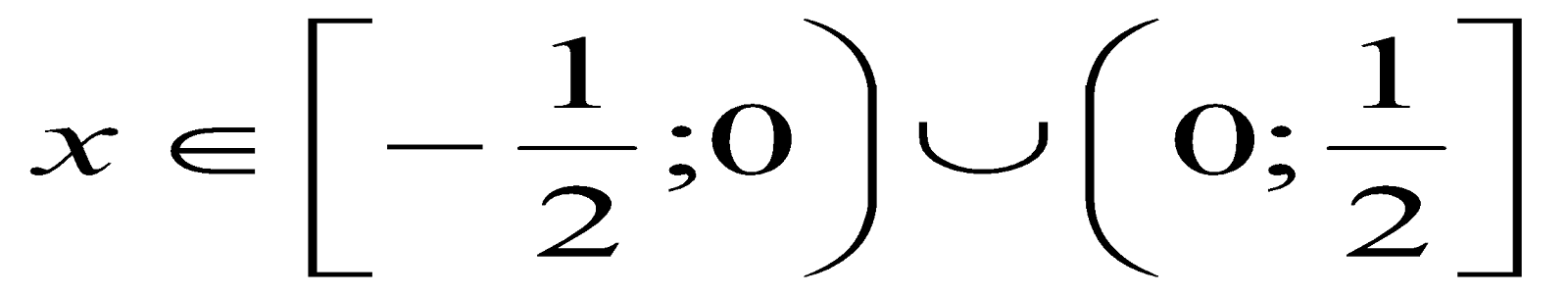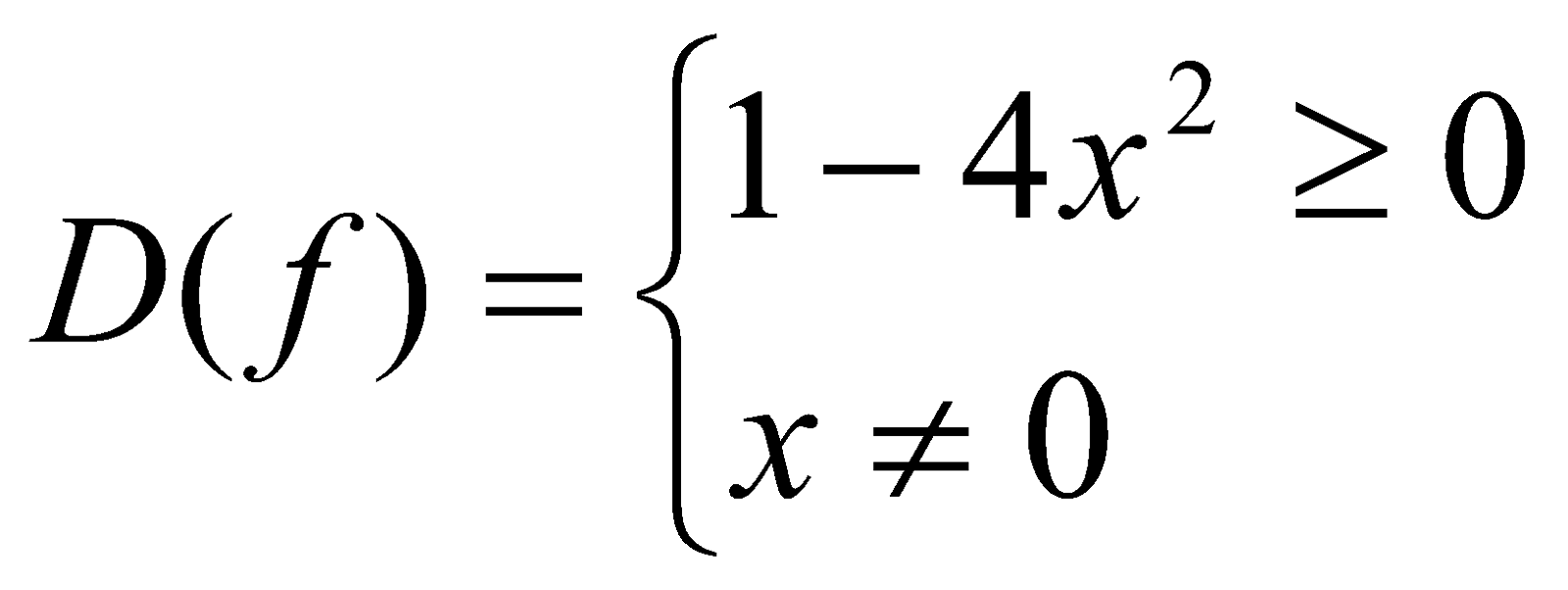3.   Determine the zeros of the function.

-satisfies the inequality of the system;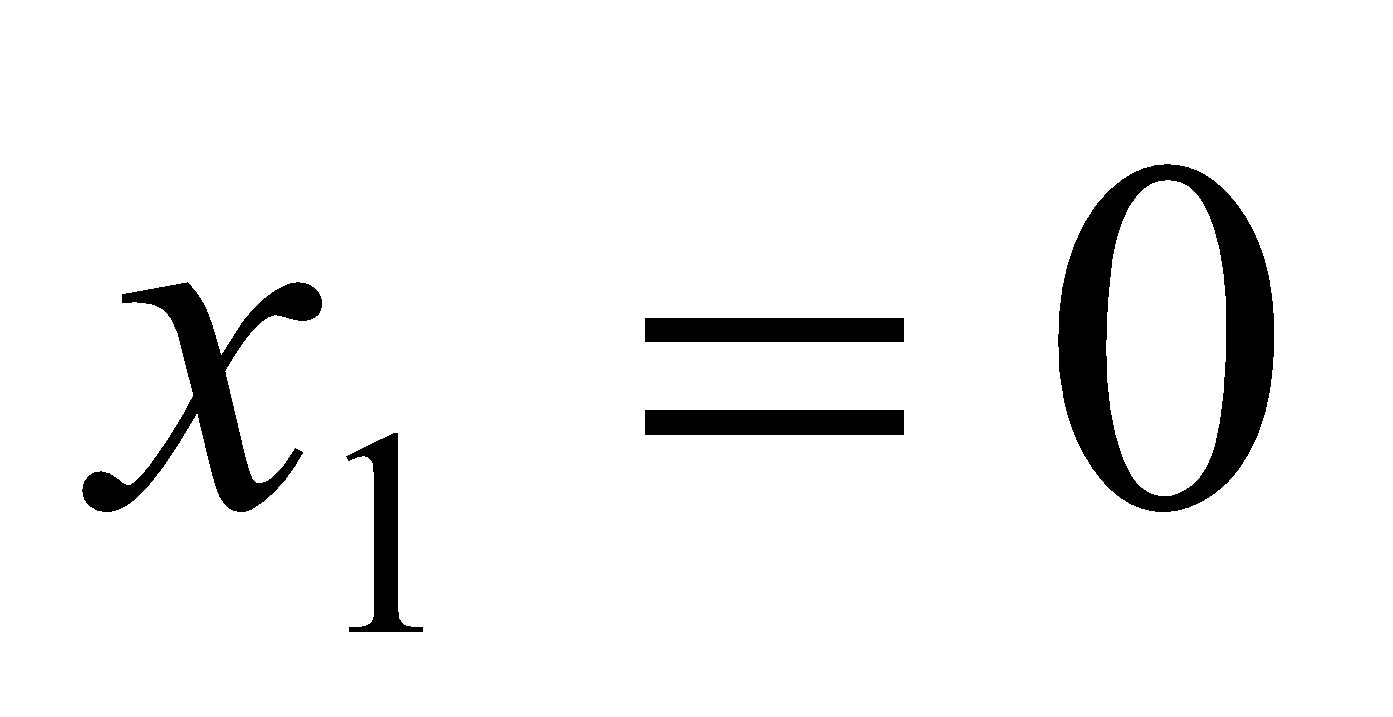-does not satisfy the inequality of the system.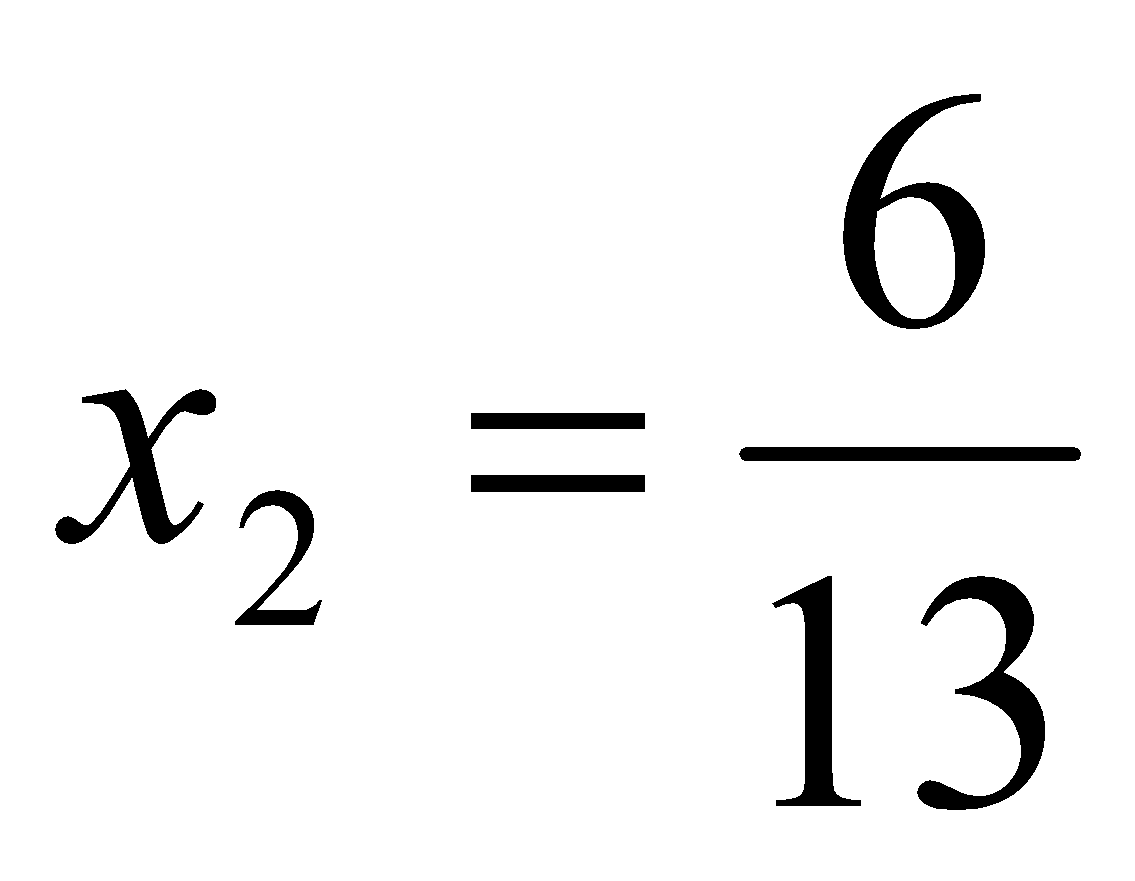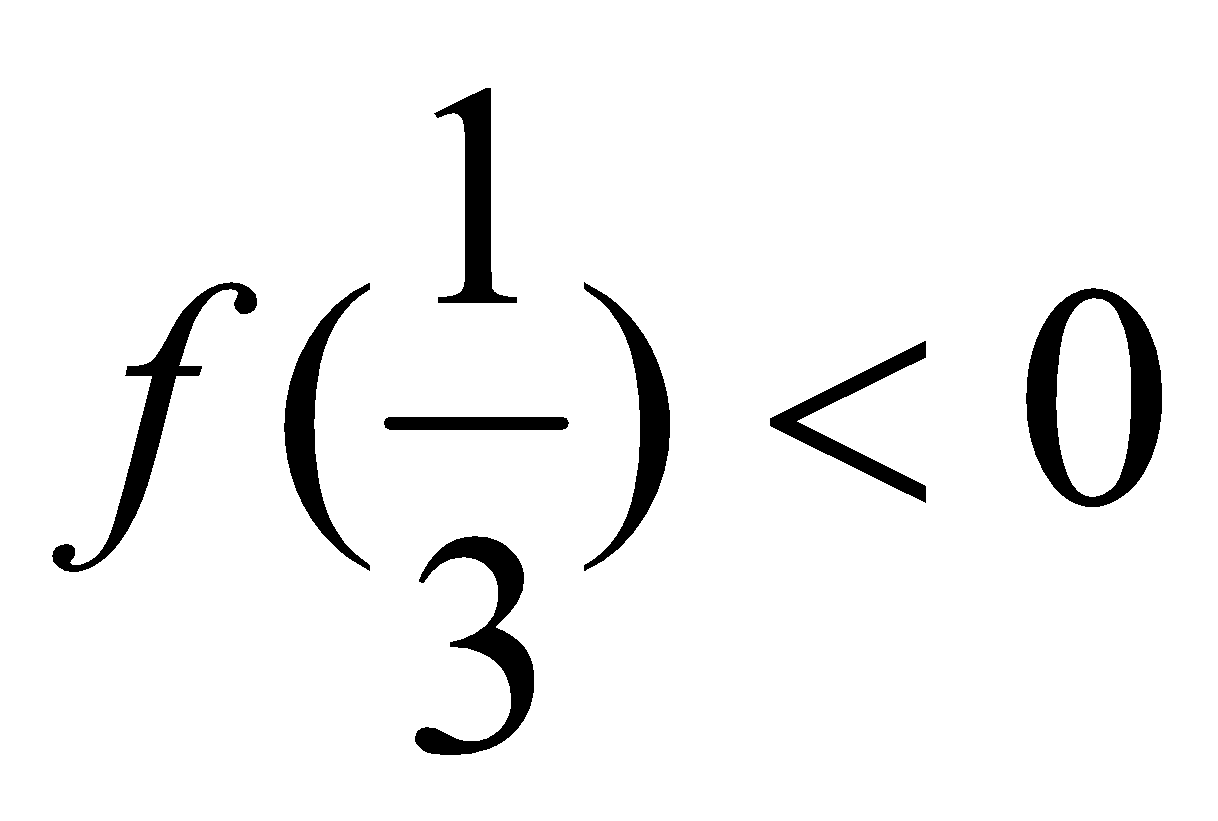4. Determine a sign on one of the interval:

The sign of the function does not change at the critical point 0 because the change of sign in the numerator «compensated" for the change of sign in the denominator.

Answer:Problem 2. Solve the given inequality: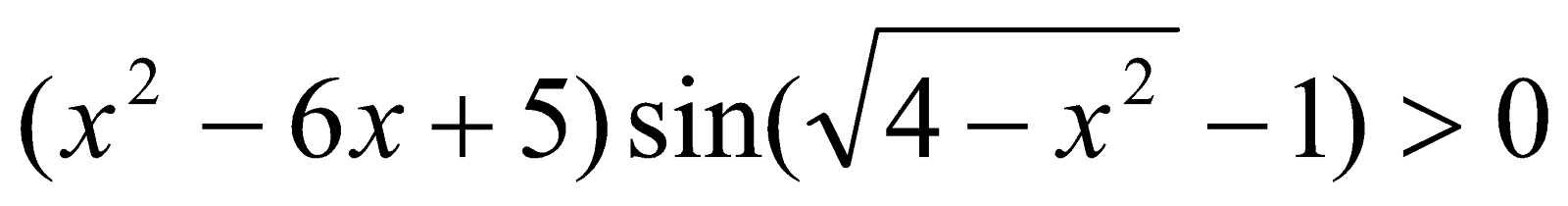Trigonometric function is presented in this inequality. It greatly complicates the analysis because of the periodicity of this function.

1.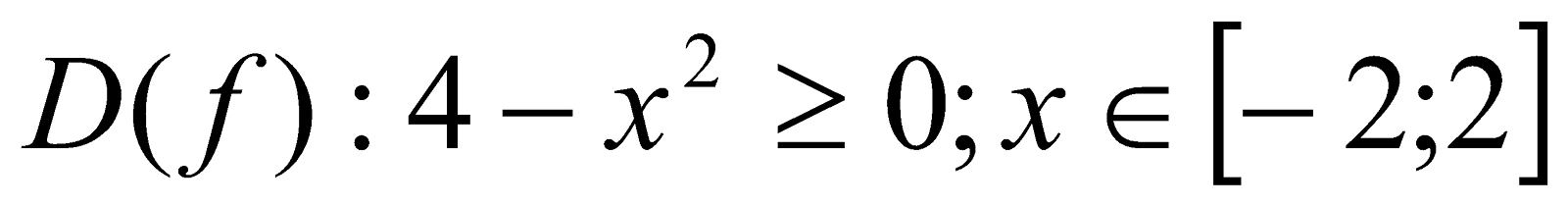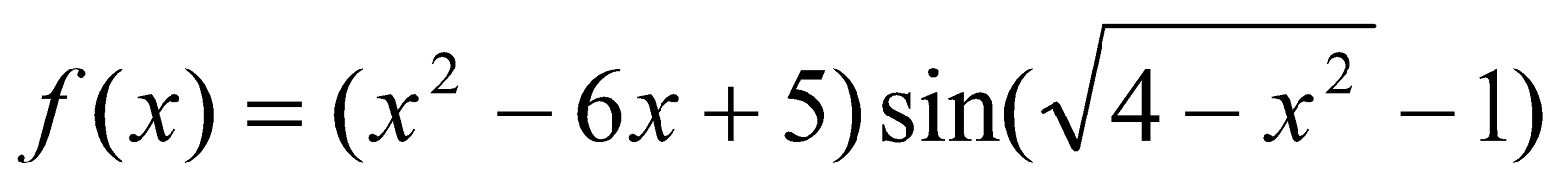3.    The zeros f(x):

Or

(This equation has solutions when n=0, 1, 2, 3, ...)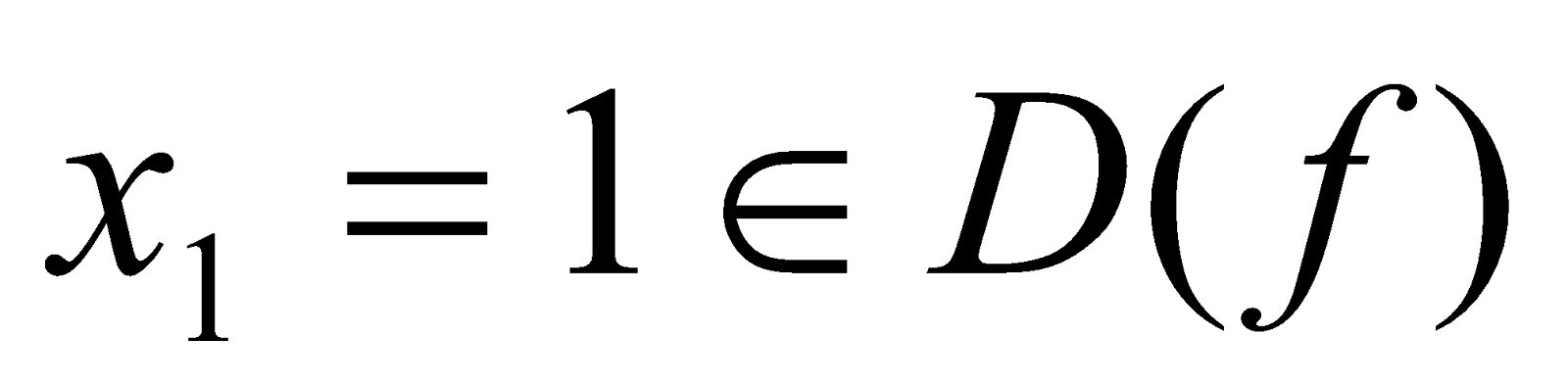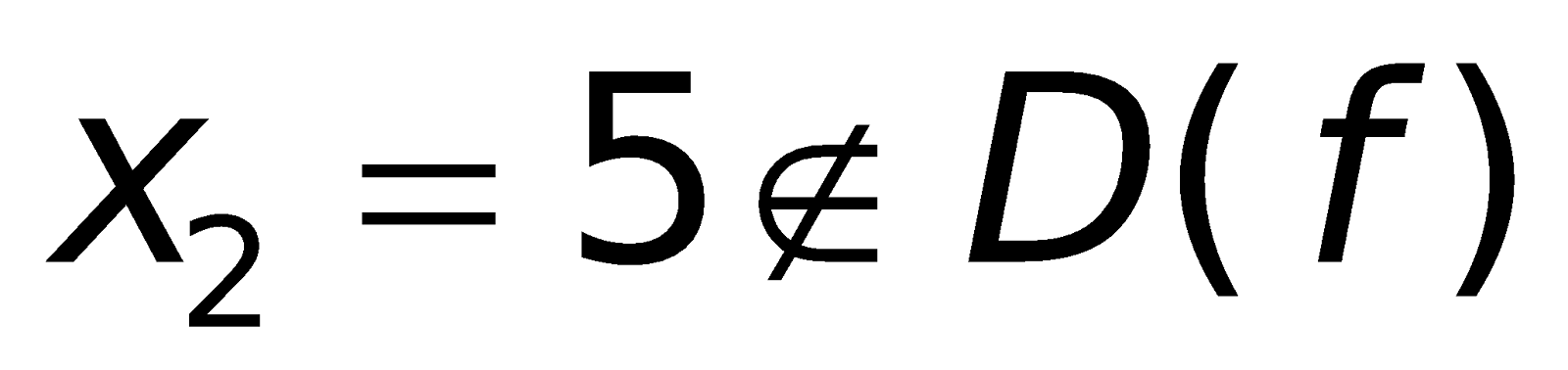4. f (0)=5⋅sin1>0

All the roots have multiplicity 1

Answer: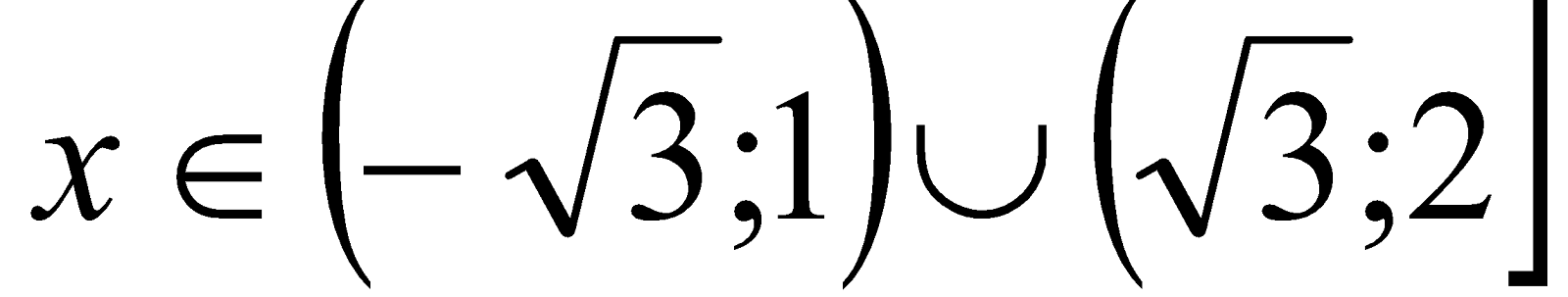Our experience shows that the targeted systematic use of the method of intervals and the accompanying use of generalizing analysis facilitates a semi-conscious motivation to the purpose (the formation of the generalized method of solving inequalities).

4.2  The requirement of balance.

The requirement of balance means that it is necessary to balance strict logical reasoning and "plausible" reasoning, which has a visually- intuitive nature. Lack of attention to this requirement contains the danger of inconsistencies between the level of mental development reached by students (as well as the predominant component of their thinking) and the teaching material used. The motivational potential of this material is weakened.

Regarding the teaching of algebra, this requirement means a broader focus on the "visualization" of analyzed. Objects based on geometric intuition to identify significant regularities of these objects. Let us illustrate this using equations and inequalities with parameters. These equations and inequalities contain in a concentrated form an infinite set of equations and inequalities. The teacher to use appropriate teaching strategies to clearly highlight the features of this method for their students. From the analysis of a some of textbooks and exploring experience of many teachers, it is understandable that the tasks within the parameters are considered episodically in the classroom without proper emphasis on a holistic perception of the generalized methods of activity. In showing how to solve these tasks teachers tend to use formal enumeration of possible values of the parameter, which leads to a large number of variants, which are difficult to analyze. This approach makes it difficult to conceptualize the parameter as a special kind of free variable whose value is given, and for many students, this causes a challenge [22, 23].

In our own practice, these equations and inequalities with parameters are considered on the visual-geometrical base. It possible to significantly stimulate the cognitive activity of the student through explicitly highlighting the most significant stages of the solution process. Let us give some examples.

Problem 3. For which values of the parameter а would represent the equation (x+1)|x-1|=a have three roots?

Analyzing with the students the graphic images of the left and right sides of the equation ion, we conclude that the first of them (the graph of the function f(x) = (x+1)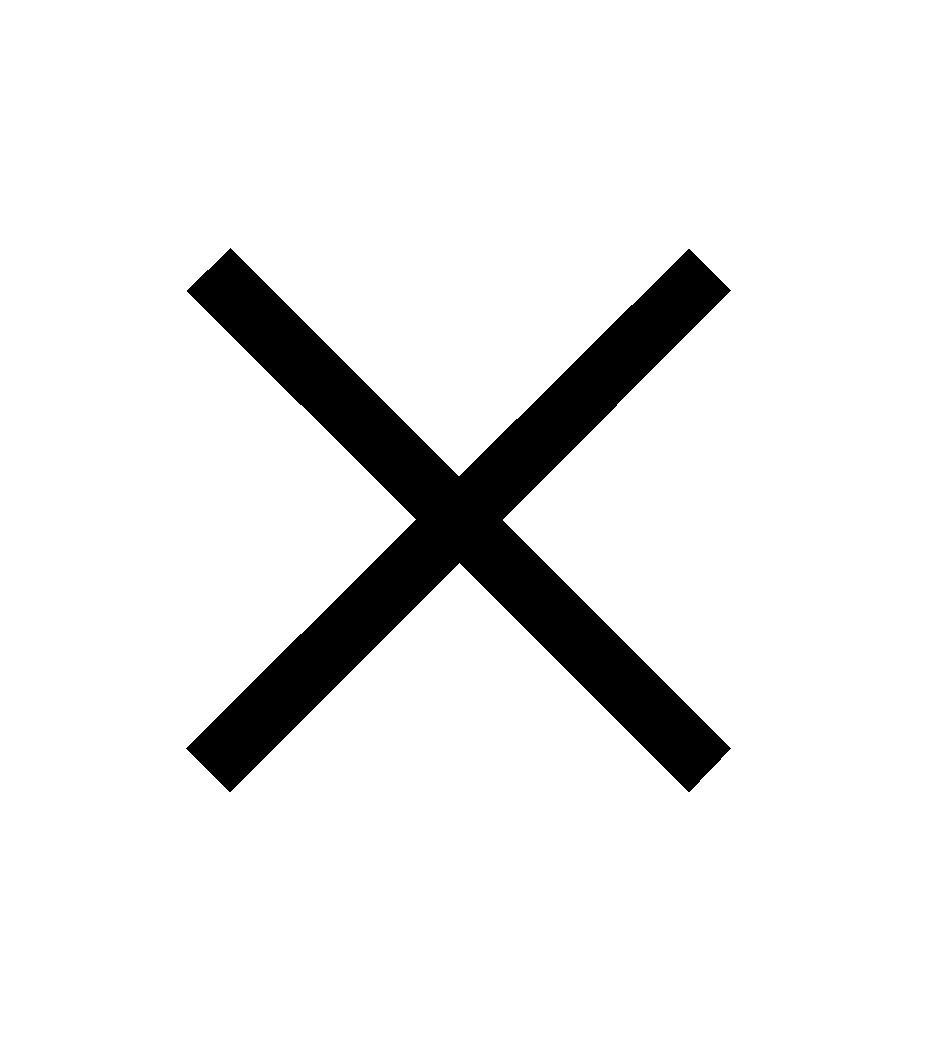|x-1|) will be fixed. The position of the second (straight line g(x) = a) depends on the value a. The abscissas of the points of intersection of these graphs will give the roots of the equation.

We rewrite the function in the following form: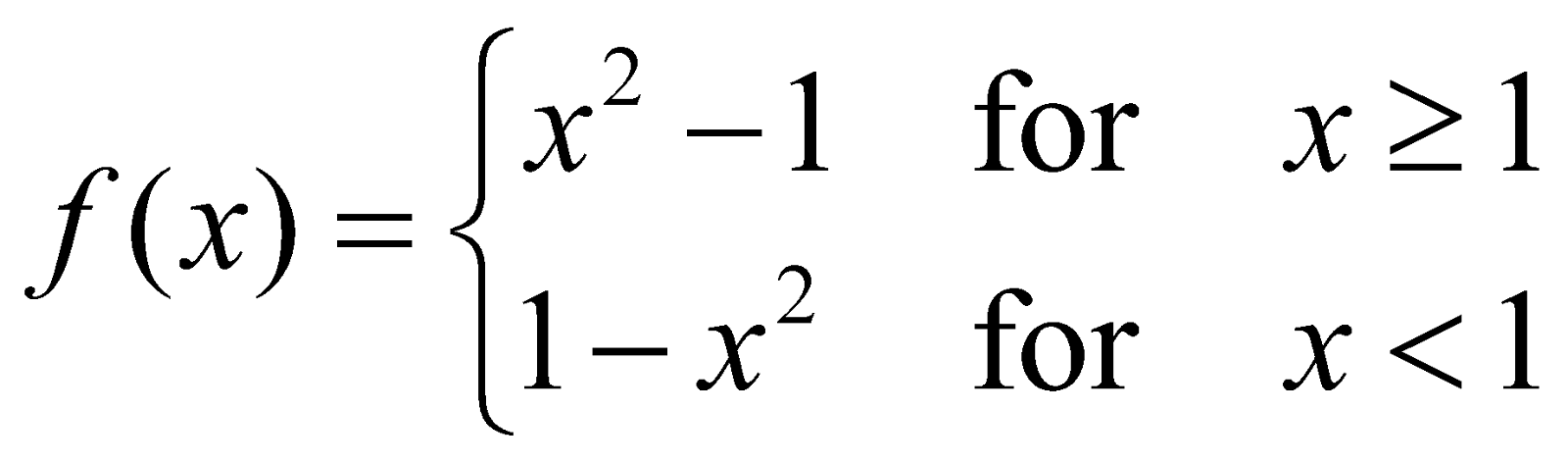Construct graphs of those functions (Fig.3).By placing a ruler parallel to the x-axis and moving it vertically, the students are convinced that the equation has three roots when:

Developing these ideas, we can consider the task which requires the construction of more complex geometric images.Problem 4. For which values of the parameter а the system has at least one solution?

Previously, we note that if а<0, the system will not have the solutions.

Let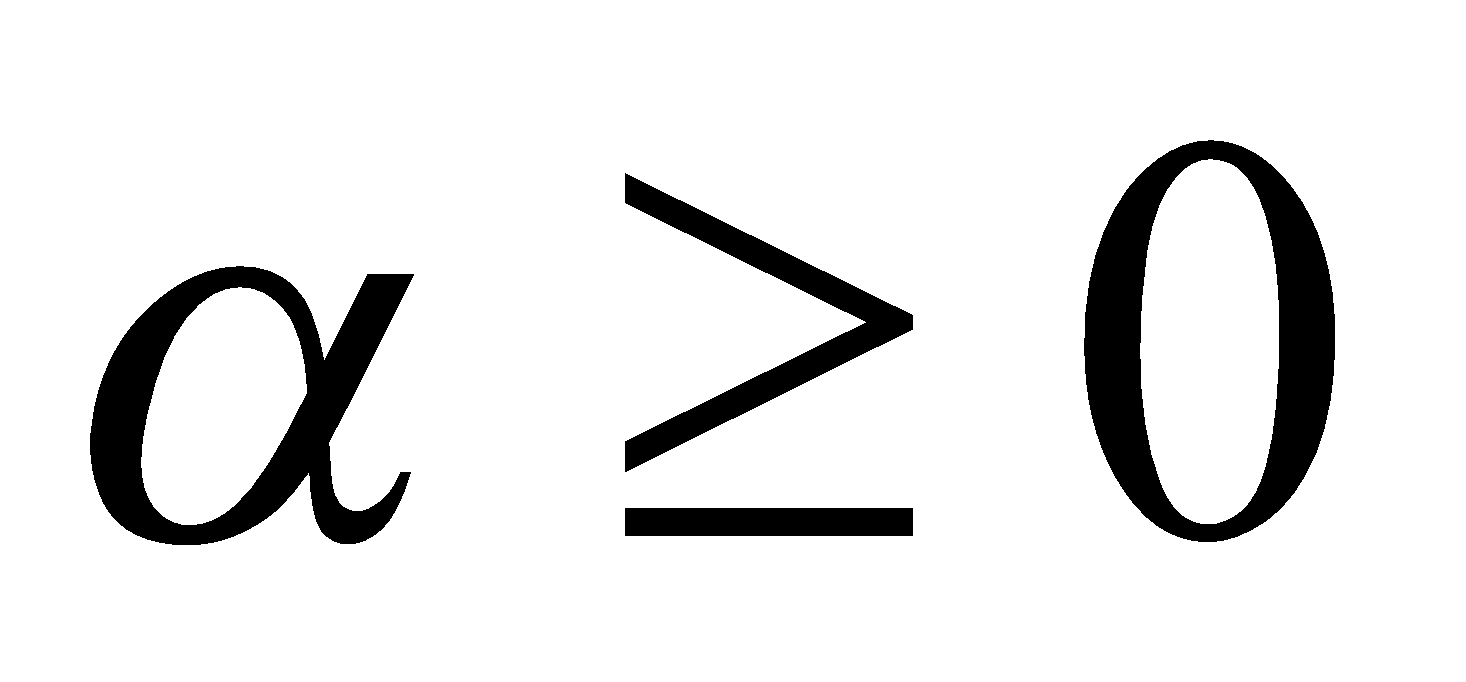. Then the first equation will be the equation of the moving circle with a radius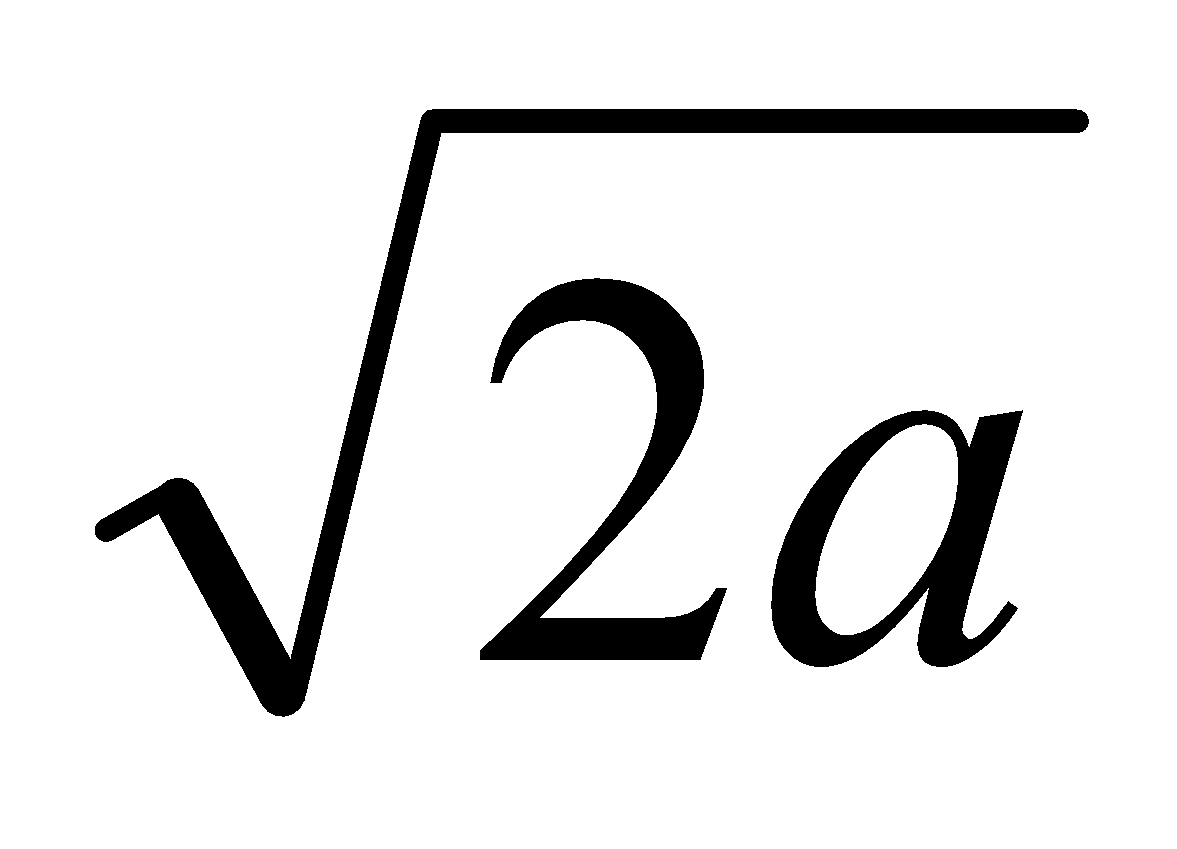. The second equation defines a fixed square with the vertices on the coordinate axes and a diagonal equal 2. From figure 4 it is seen that for the intersection of the square and the circle, its radius should be within: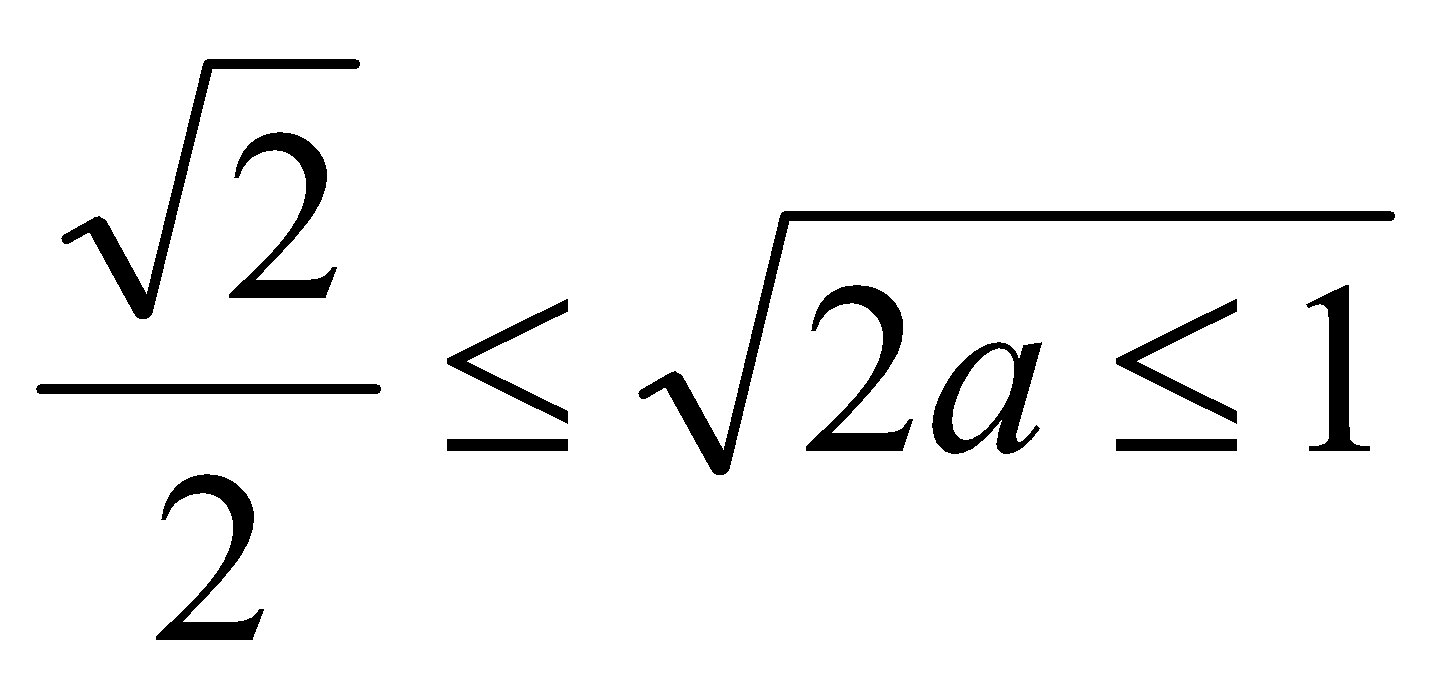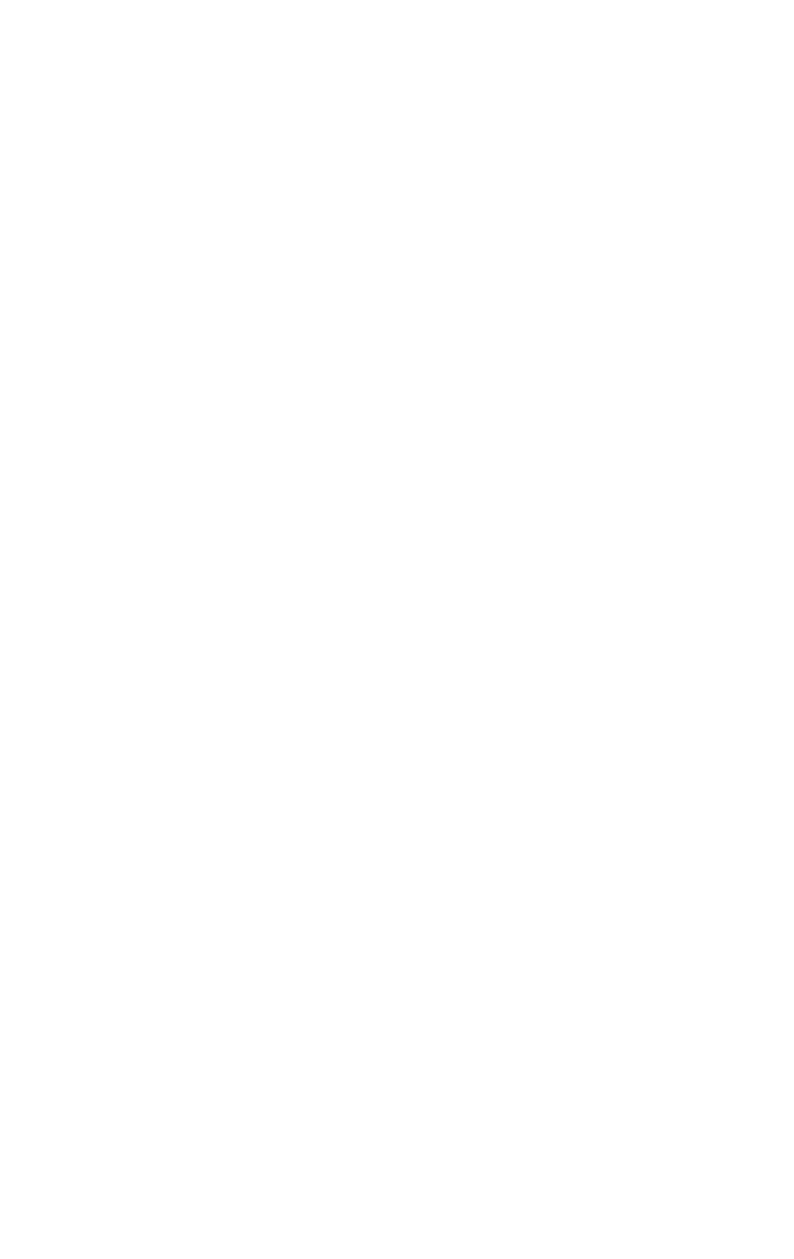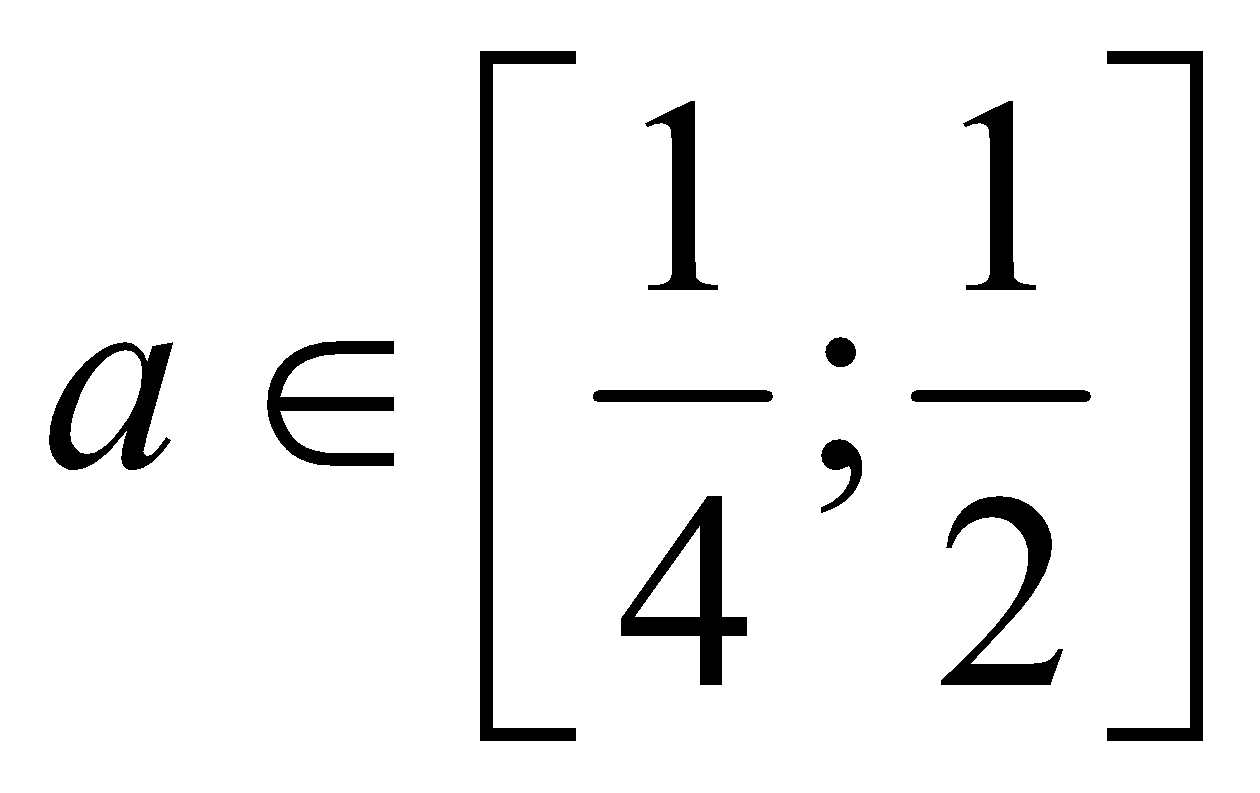4.2  Requirement of nonclosure

The requirement of nonclosure as an object of incomplete study permits expansion and replenishment. Students will explore generalizations, going beyond the topic or section of the course. That will allow demonstration of further potential for the development of mathematical material and the actualization of the motivation of anticipation [11, 19].

For example, continuing to discuss with the students about algebraic equations and inequalities with parameters, useful to consider the inequality of the following type.

Problem 5. Solve the given inequality:

In this inequality the separation of free variables а and x, as in previous cases, is not possible. Therefore there is a need to introduce (not quite explicitly) a new concept of the function of two variables for students.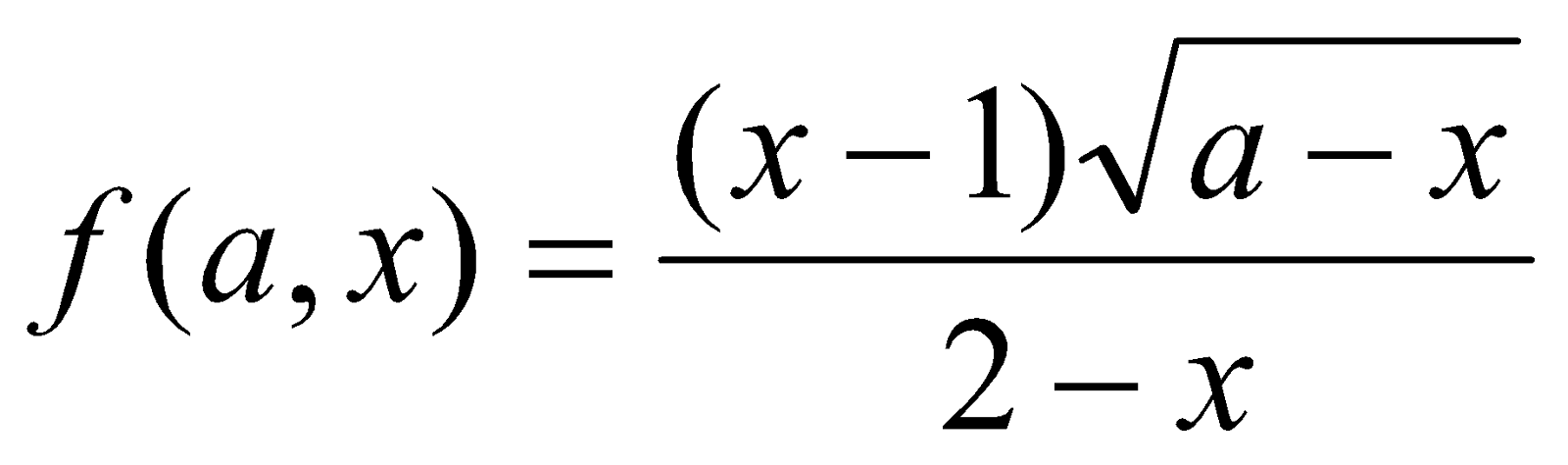.

Apply the interval method :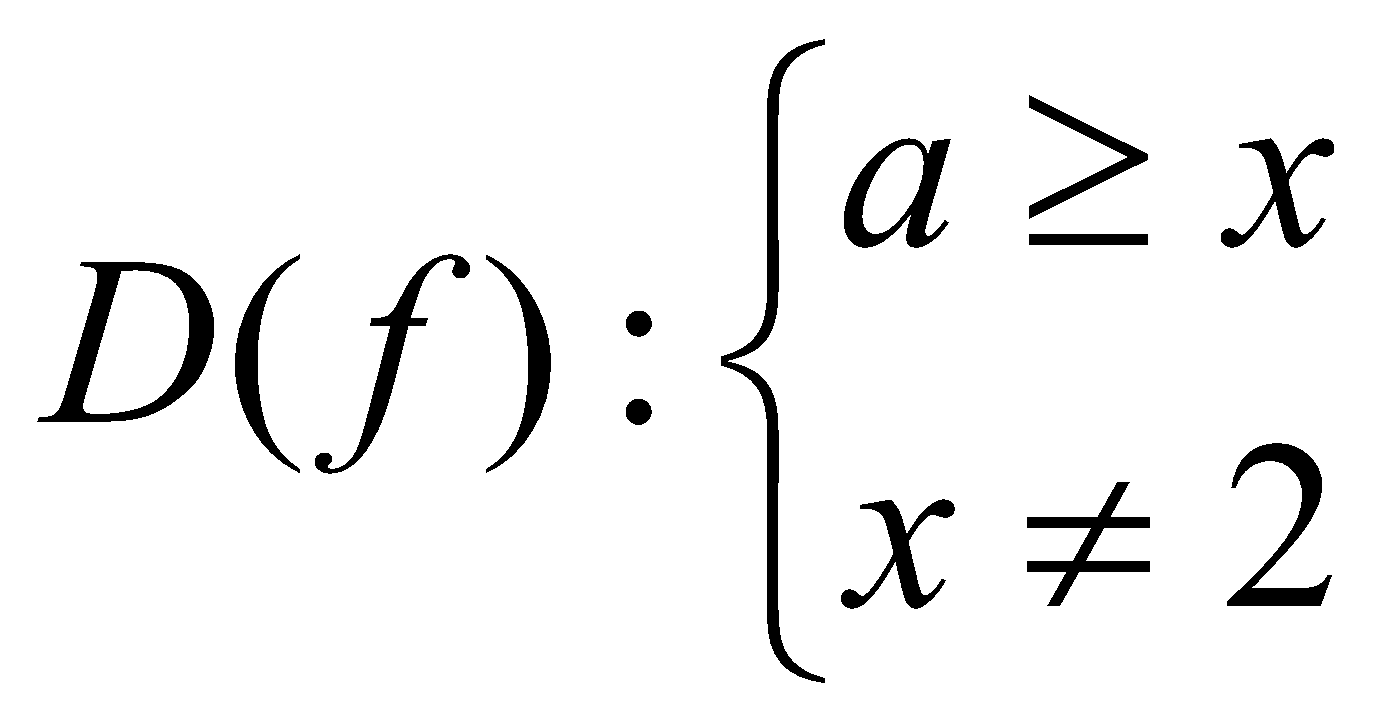The zeros: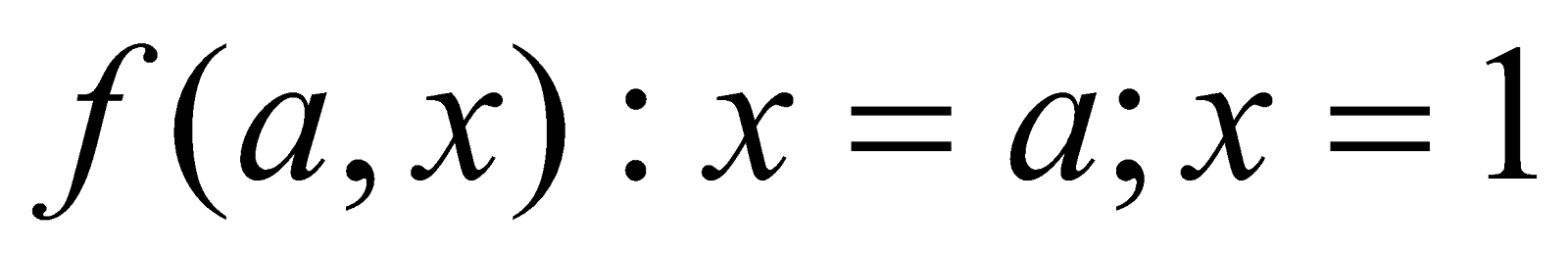.

Lines (х=1, х = 2, х = а) will implement the role of the critical points for this function, relative about the coordinate plane хОа (Fig.5). Of the three areas, which are above the straight line x=a, select the area that satisfies the initial inequality, considering that f (a=2, x=0) <0. Note that all the "critical points - straight lines" have multiplicity 1. Therefore, it is sufficient to determine the sign of the function in one area only. Moving a ruler parallel to the axis OX from down to up the students on the visual-intuitive level obtained the following result:a) for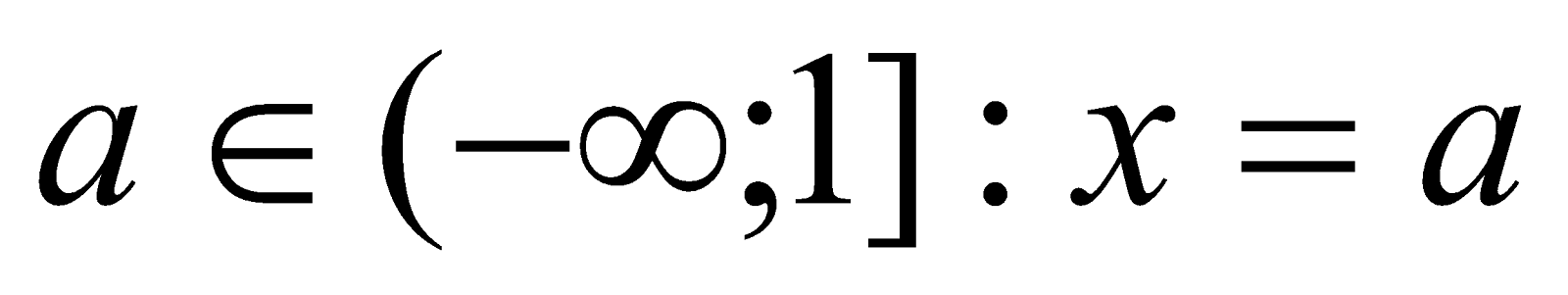; b) for; c) for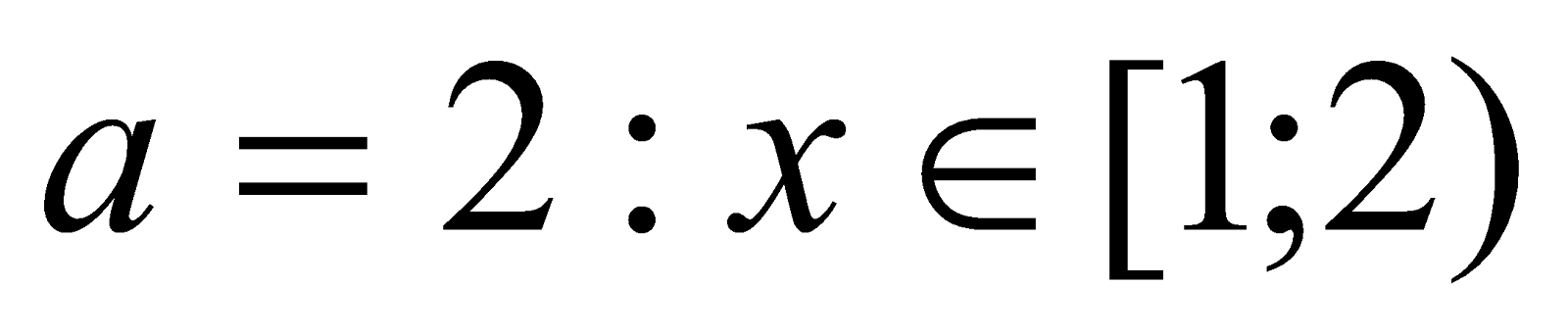; d) for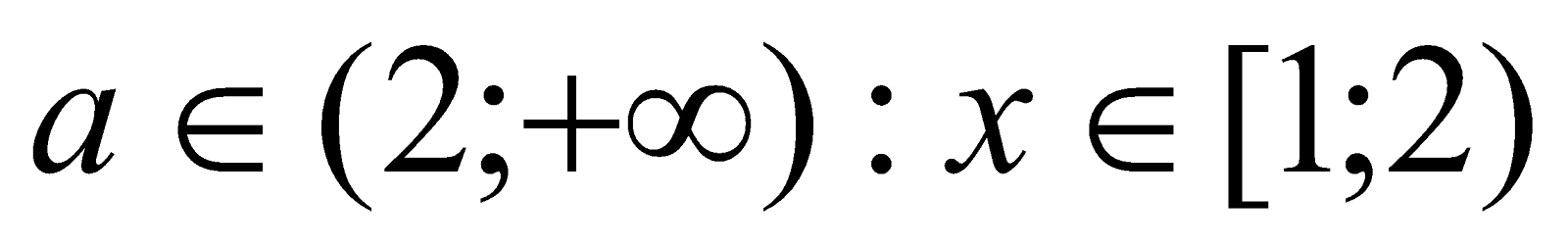and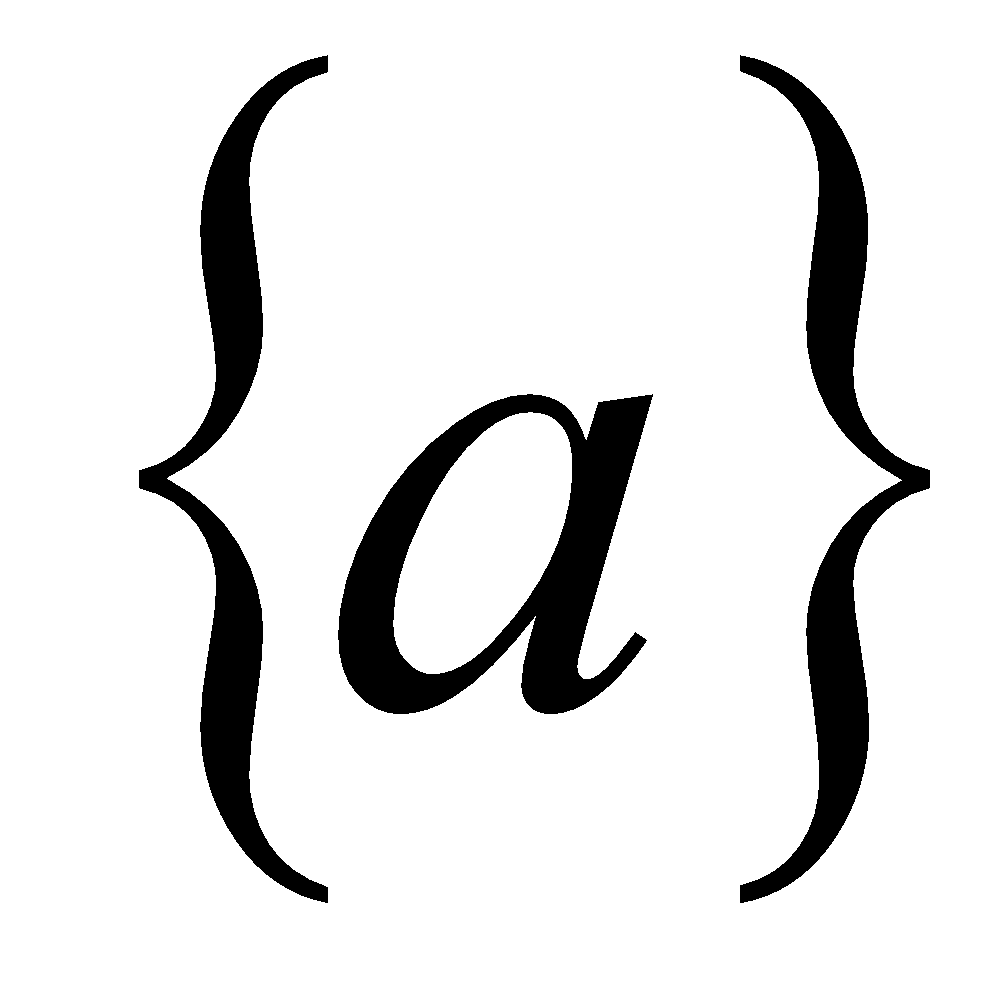Performing this task does not involve a significant change in the algorithm of the method intervals, but at the same time reveals the possibility of substantial enrichment of this way through the generalization of mathematical material. At the same time, students assimilate the generalized idea, anticipating one of the directions of its further development.

4.3  The Requirement of variability

The requirement of variability suggests the need to create conditions for a conscious choice of the most appropriate strategy and tactics in students’ search activity. The fact that "a solution can be the internal state of the individual only when it is the result of their own choice" is important [14, 16,17]. The implementation of conscious choice implies the intention and the ability of students to look at the situation "from the other side," i.e. go into another "cognitive system of coordinates." Accordingly, the teacher should give the tasks with the possibility of alternative approaches to the solution, evaluating their effectiveness. As indicated by Menchinskaya , a teacher creates a need to improve the used methods and to replace less rational methods by those which are more efficient.

Consider absolute value equations and inequality. School practice shows that the formal approach is used in teaching this topic. It leads to repetitive attempts to use the definition of module even in cases where it beside the point and purpose. For example, when students solved equations with Absolute value equations or modulus equation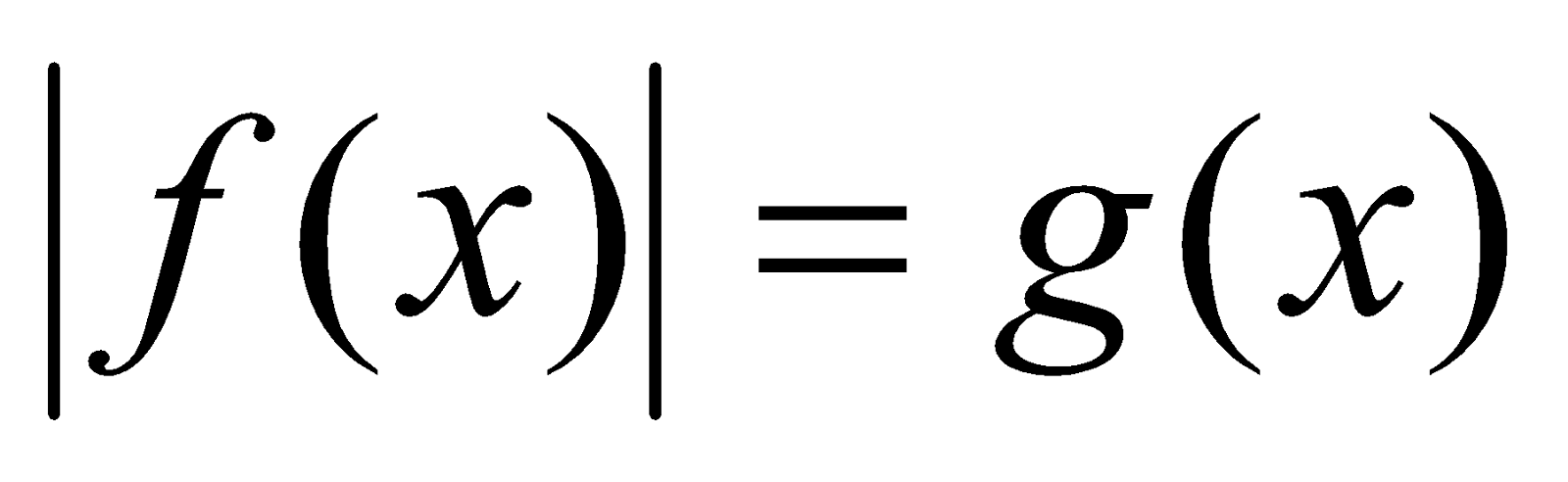they used a template and obtained the following: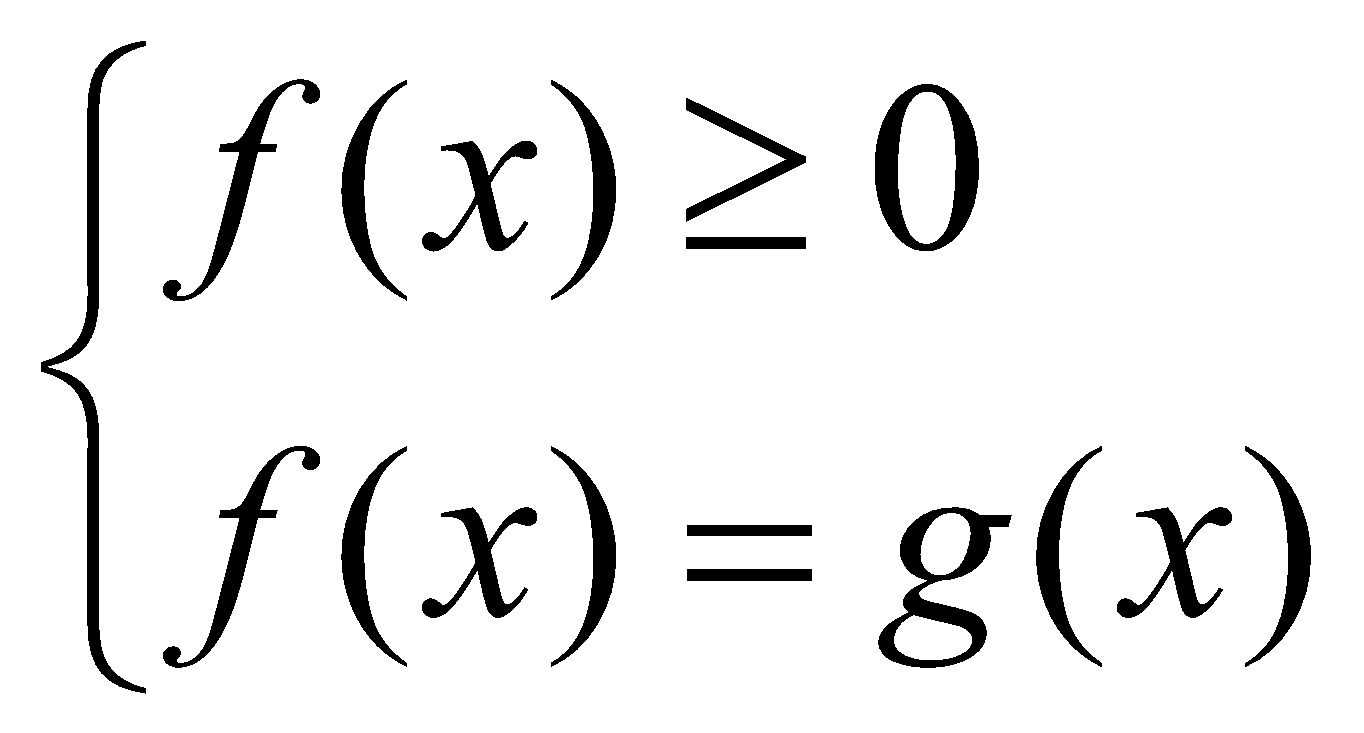or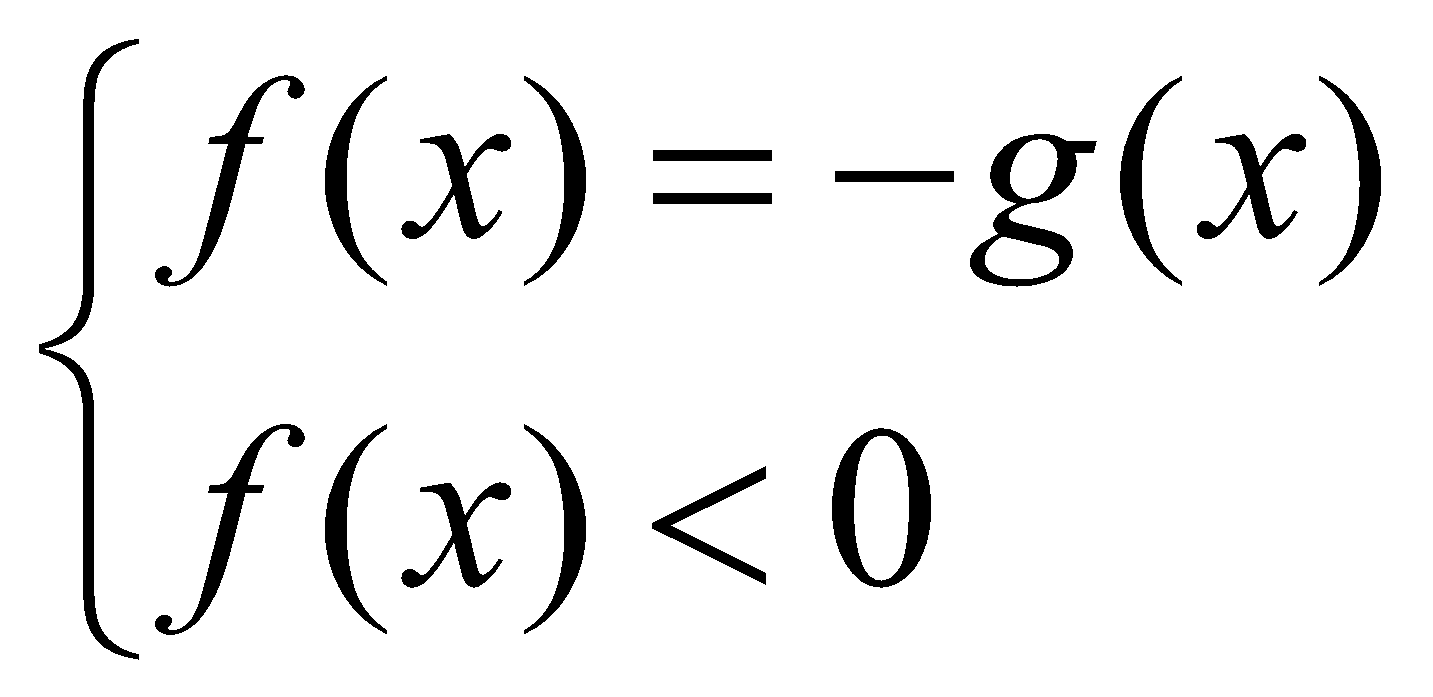However, in some cases, function f (x) has a much more complicated form than function g (x), and therefore it is rational to replace the above- recorded set of systems with the equivalent set of: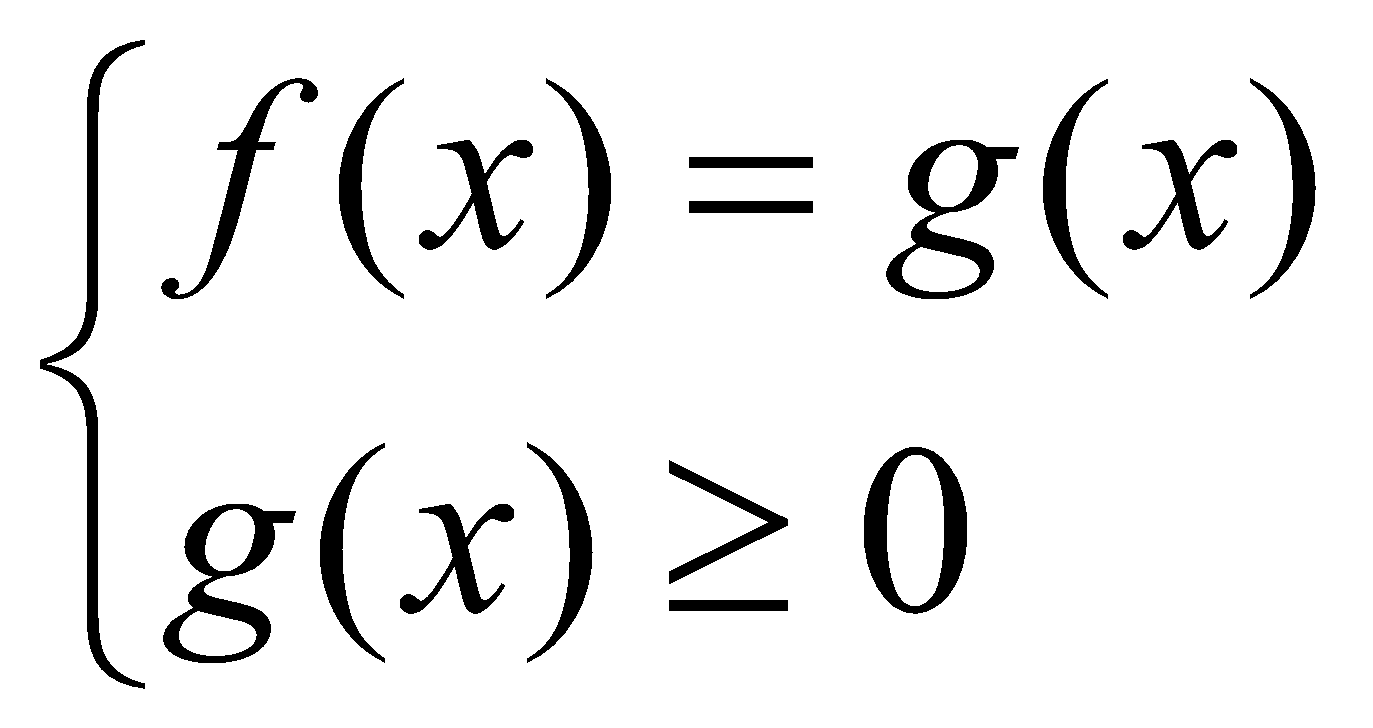or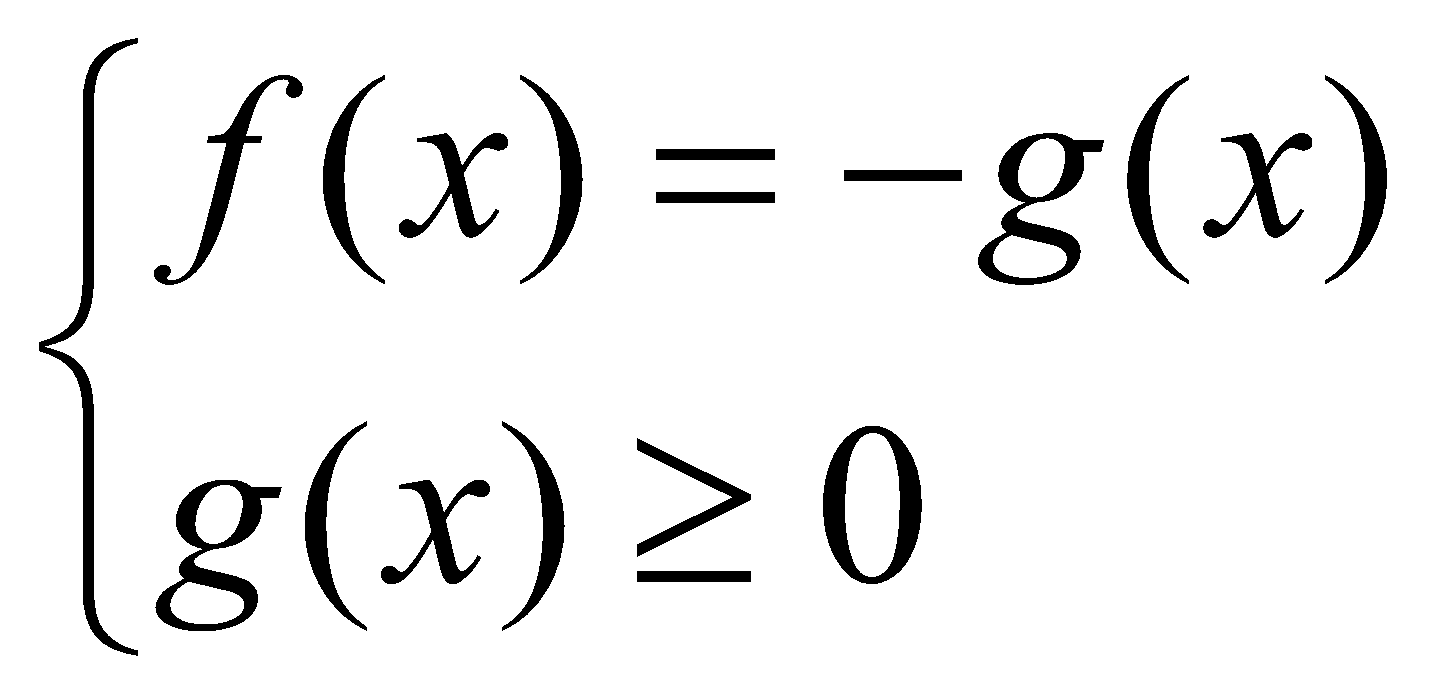.

Let us illustrate such a replacement by a concrete example.

Problem 6. Solve the given equation: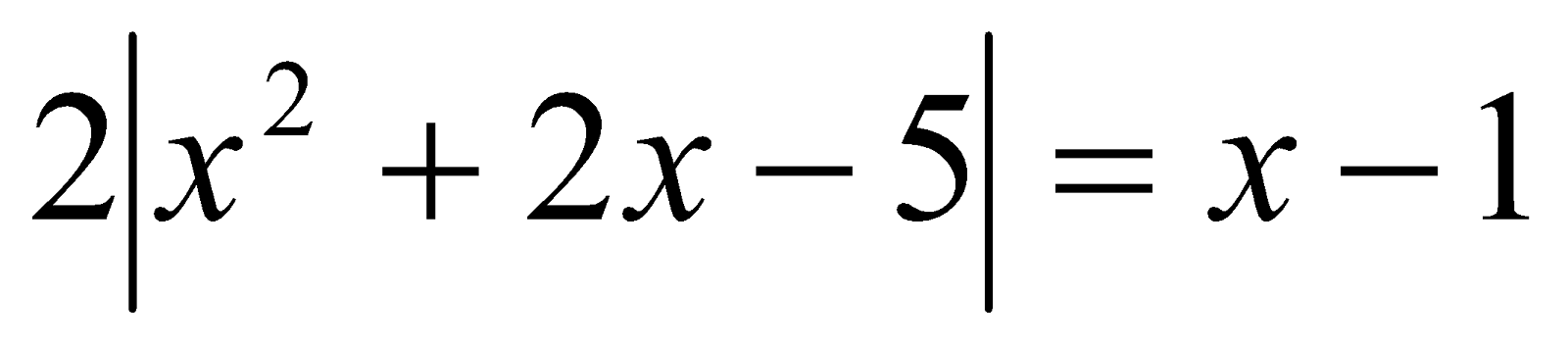We choose from the two functions one which has a simple form (patently that it is g (x) = x-1). Create the following set of systems: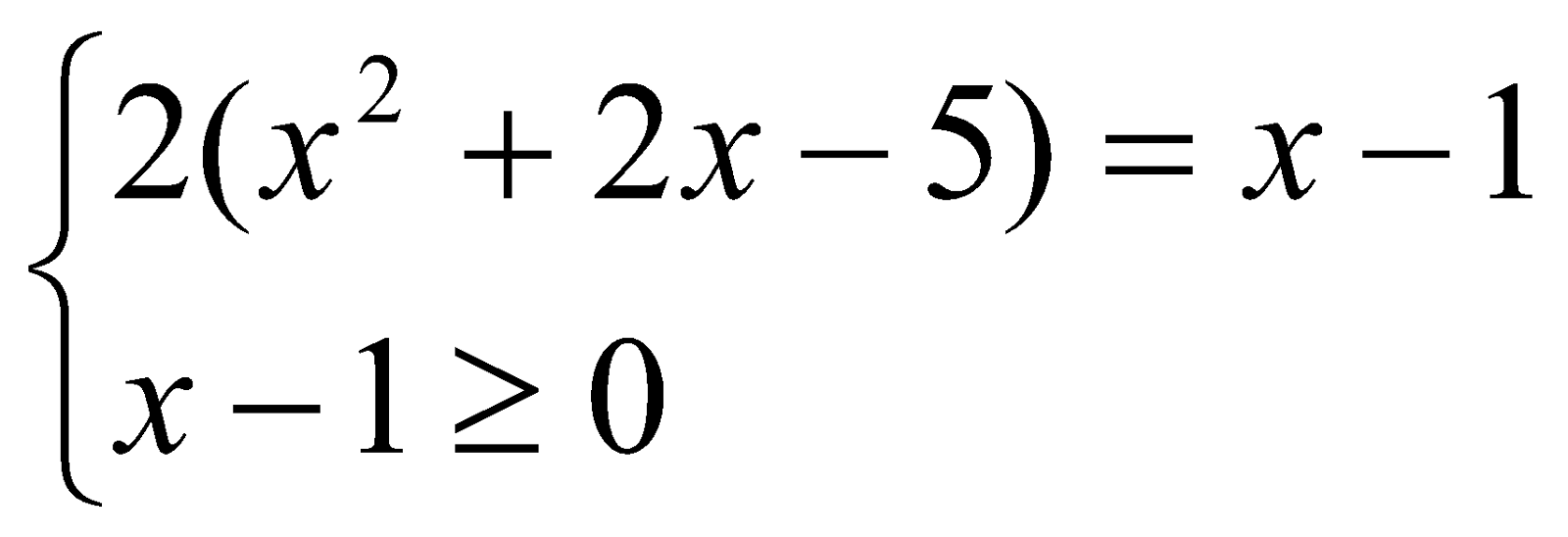orAnswer: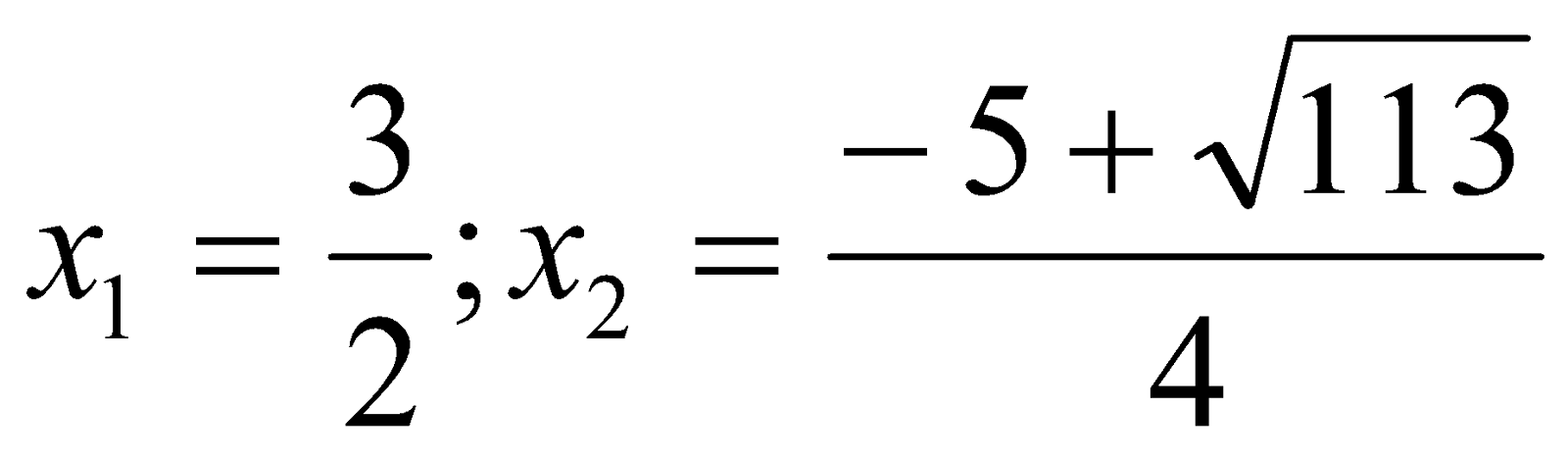As homework students should be asked to solve this equation in their habitual way and to compare the effectiveness of both solutions.

4.4  The requirement of the "struggle of opposites"

The requirement of the "struggle of opposites" brings to the fore the need to show the students the possibility both the imaginary and the real contradictions of content of math course, including the consequences of certain limitations of the mathematical method, incorrect reasoning and the imperfections of our intuition and imagination. This requirement is a "dialectical counterweight" to the requirements of 1 and 3, showing that any method of reasoning (evidence based or empirical-intuitive) has a certain limited functioning range. When the students become aware of this range, they tend to clarify the features of the application of that method, and to revise traditional representations, moving them to a higher-level thinking [7, 10, 18].

For example, studying the graphical method of solving equations and inequalities, students may start to believe in the universality of this method and the inappropriateness of the analytical method. To show the fallacy of this point view the teacher can offer students the following problem.

Problem 7. Is it true, that the equation: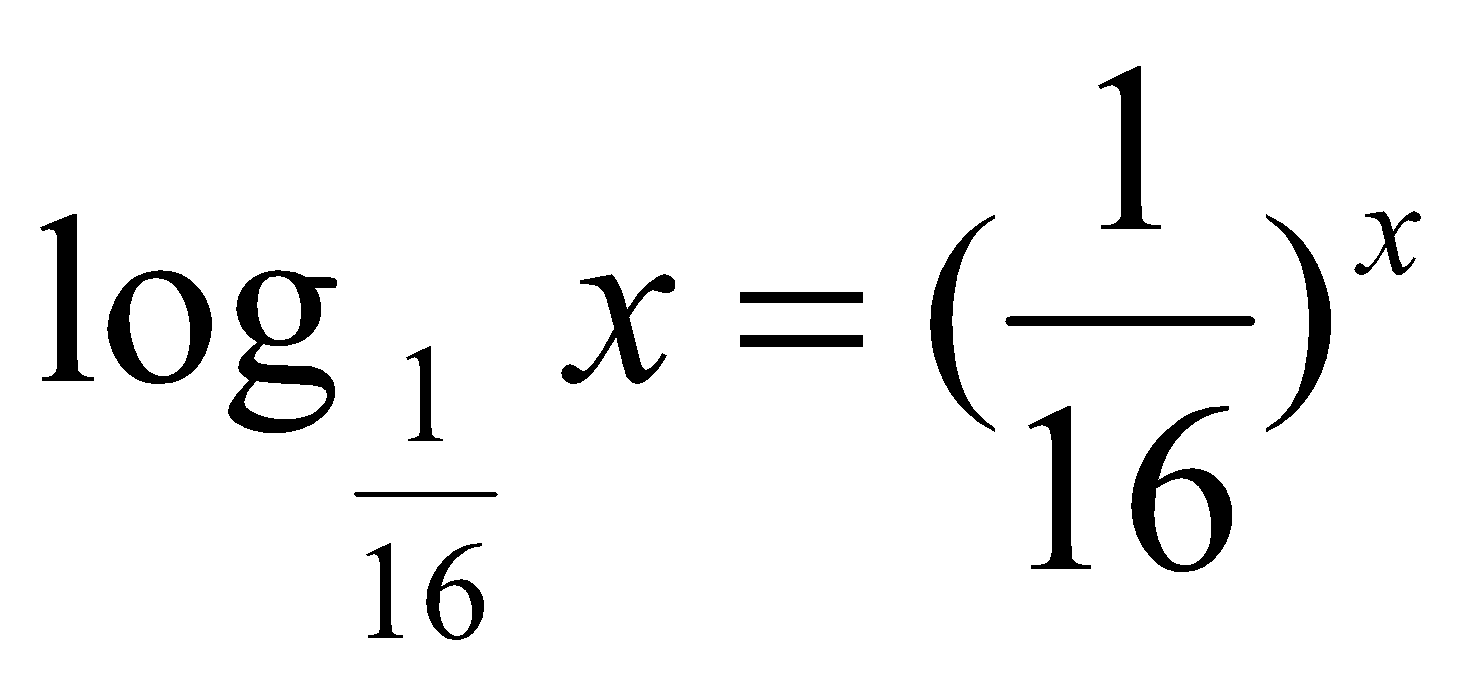has exactly one root? [12, p. 250].

Note that in this case the task itself implicitly provokes students to estimate the number of intersection points of the graphs. When this graph is constructed (Fig. 6), students can conclude that they intersected in one point located on the bisectrix of the first and third coordinate angles. However, there are two additional roots if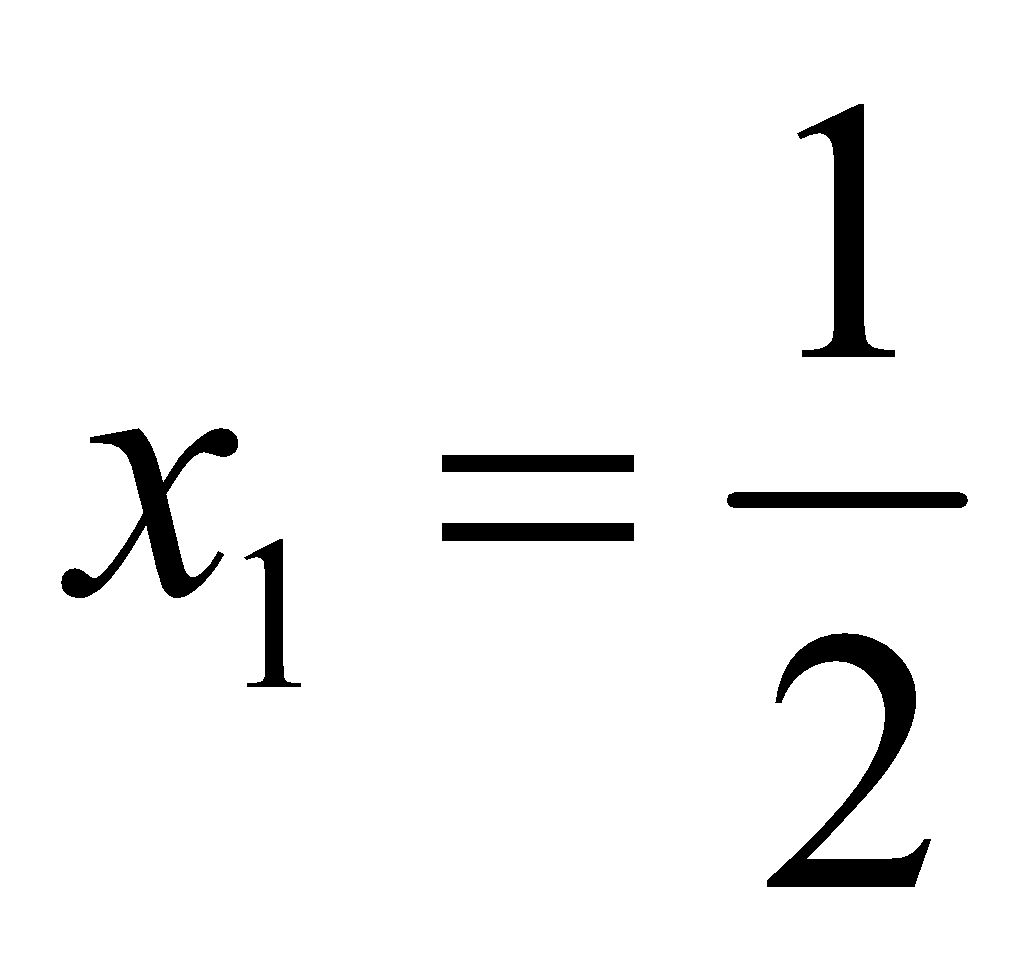and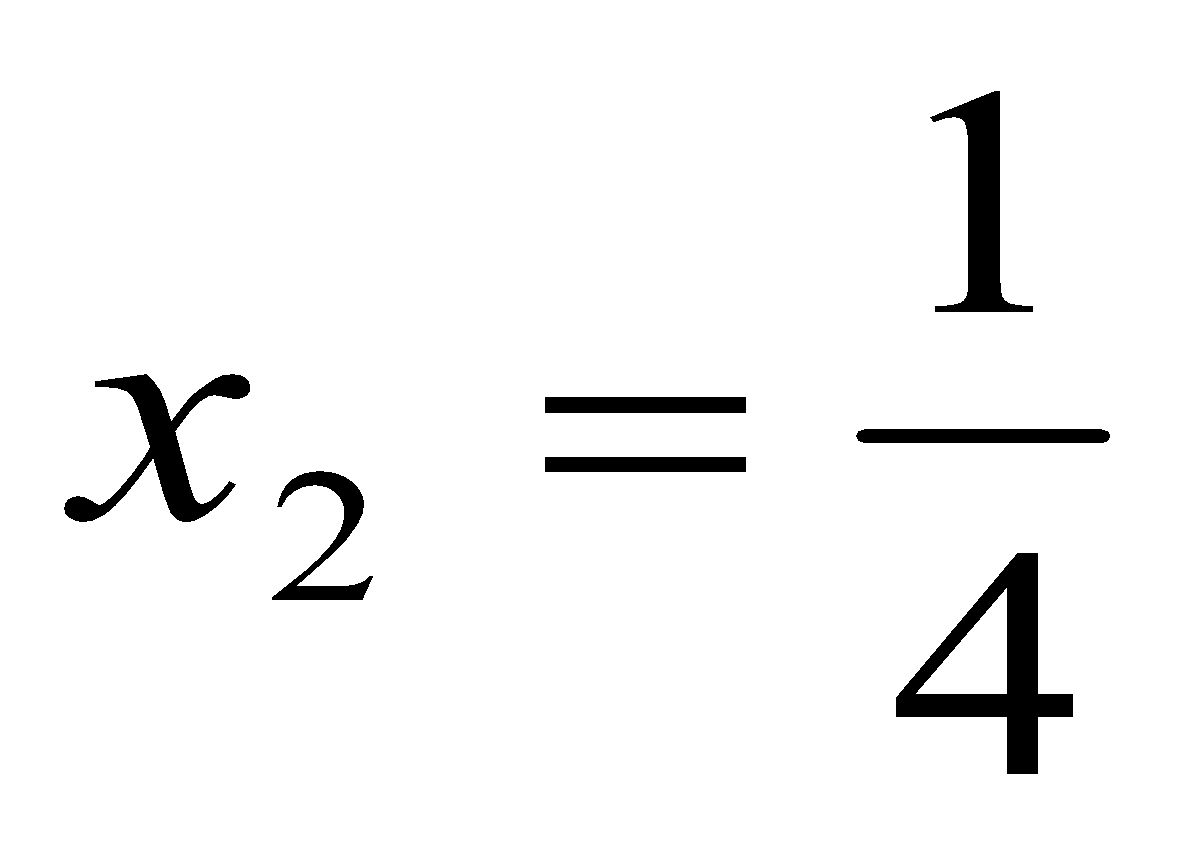is substituted in the initial equation.

This solution demonstrates to the students the imperfection of a solely graphical approach and motivate them students to explore a wider validity of solutions.

4.6  The requirement of openness

The requirement of openness means the ability to transfer ideas and techniques from specific sections to other sections of school mathematics, which initially appear to be "subjectively distant" from each other. Thus the beginning of the overt task contains a disguised form of another task, the features of which are suddenly opened up. A. Esaulov described this situation as, from the original problem, "scooping out new content and unknown properties" to reinforce its potential and actual significance for the students [21, p.24]. To illustrate the latter provision we shall consider derivative from an existing algebra course. The use of this material in schools lead to the disclosure of the geometric and physical meaning of the derivative and the construction of graphs of functions on the basis by their preliminary investigation. There may be semantic barriers which will slow down the readiness of students to use all the resources and potential in this section of the school of mathematics. To extend the application of differentiation within the existing subject content, a teacher can to offer to students tasks for proof.

Problem 8. Prove the inequality: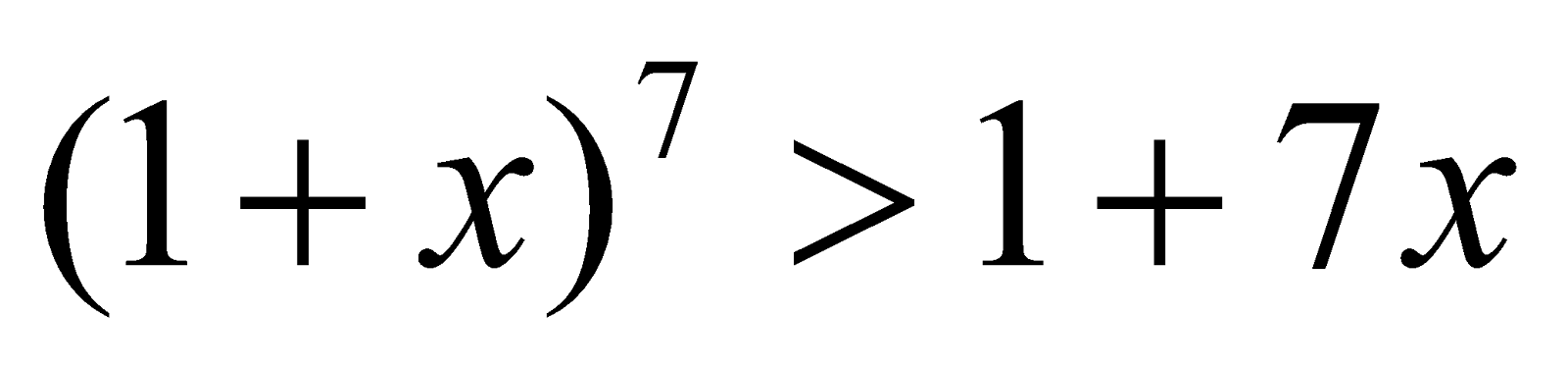if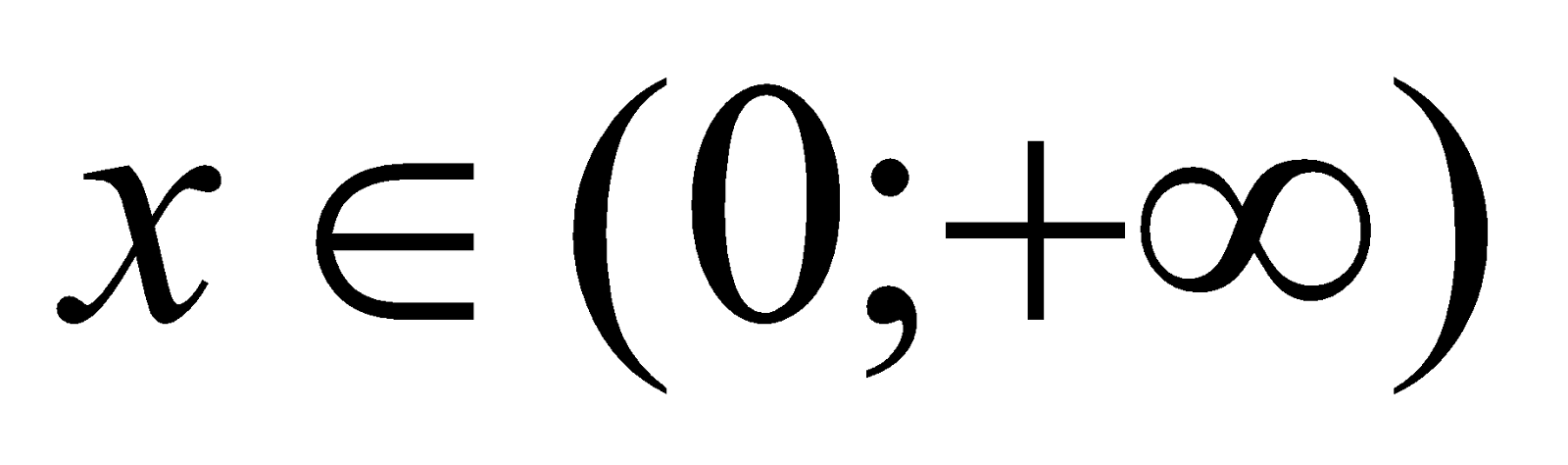In most cases, students attempt to solve similar tasks "blindly," carried out a large number of algebraic transformations (usually unsuccess- fully). They are trying to transform the inequality to a form suitable for use via the interval method. Meanwhile, the teacher’s proposed derivative as a way of exploring a function at specific intervals can radically change the situation, causing feelings of surprise and interest in students.

1. Consider the function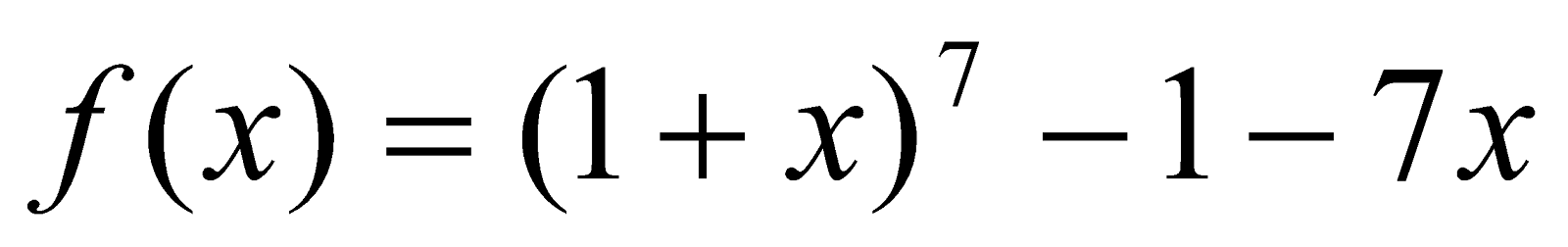and explore this function at the interval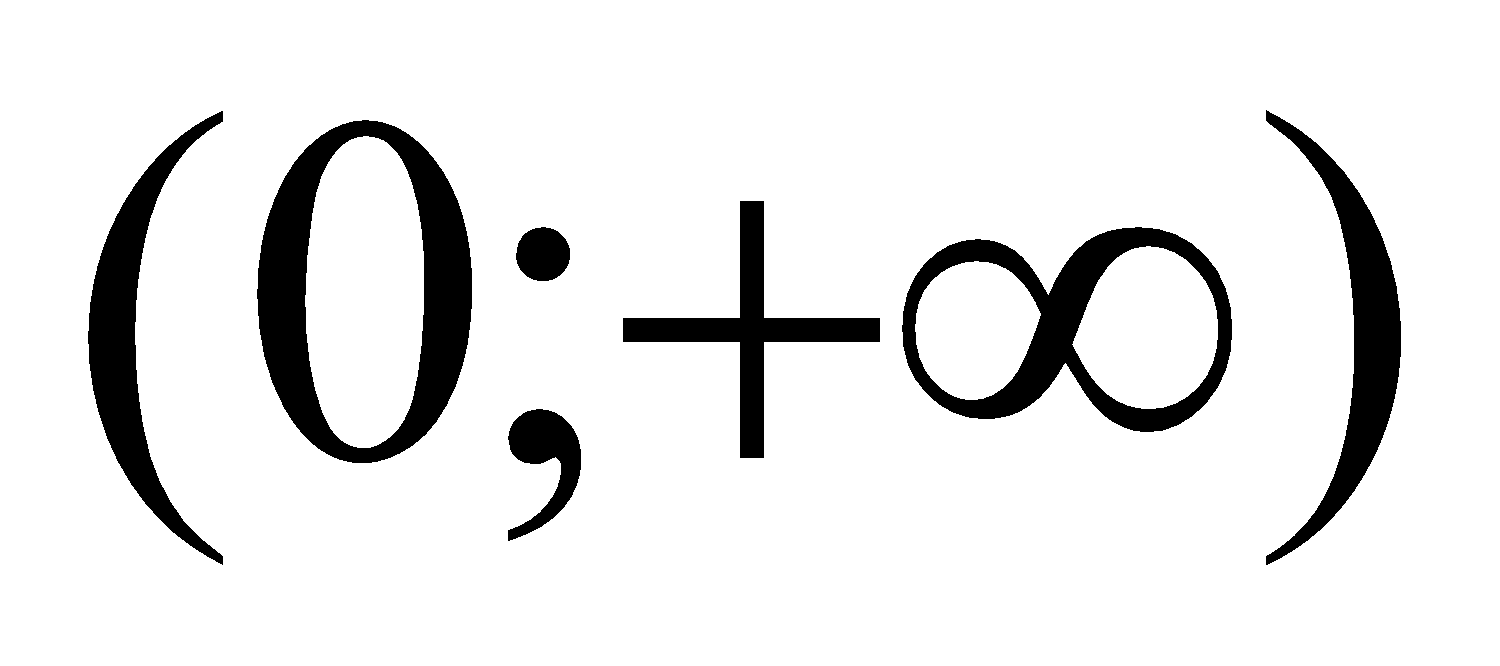;

2.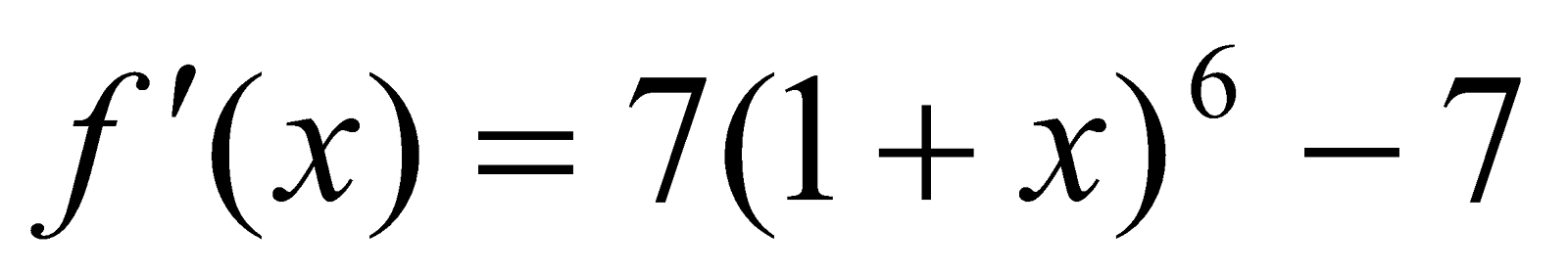;

3. Critical points:

4. Define the sign of the derivative at the interval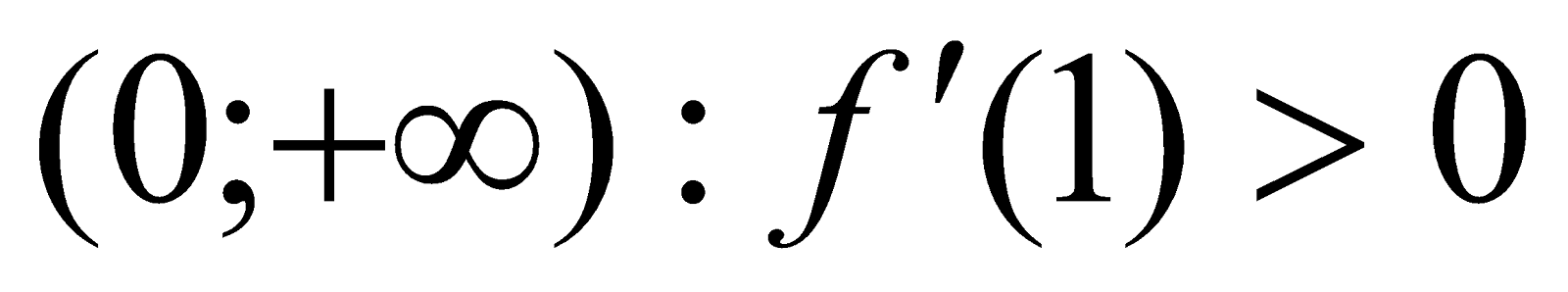5. The function f (x) increases ifand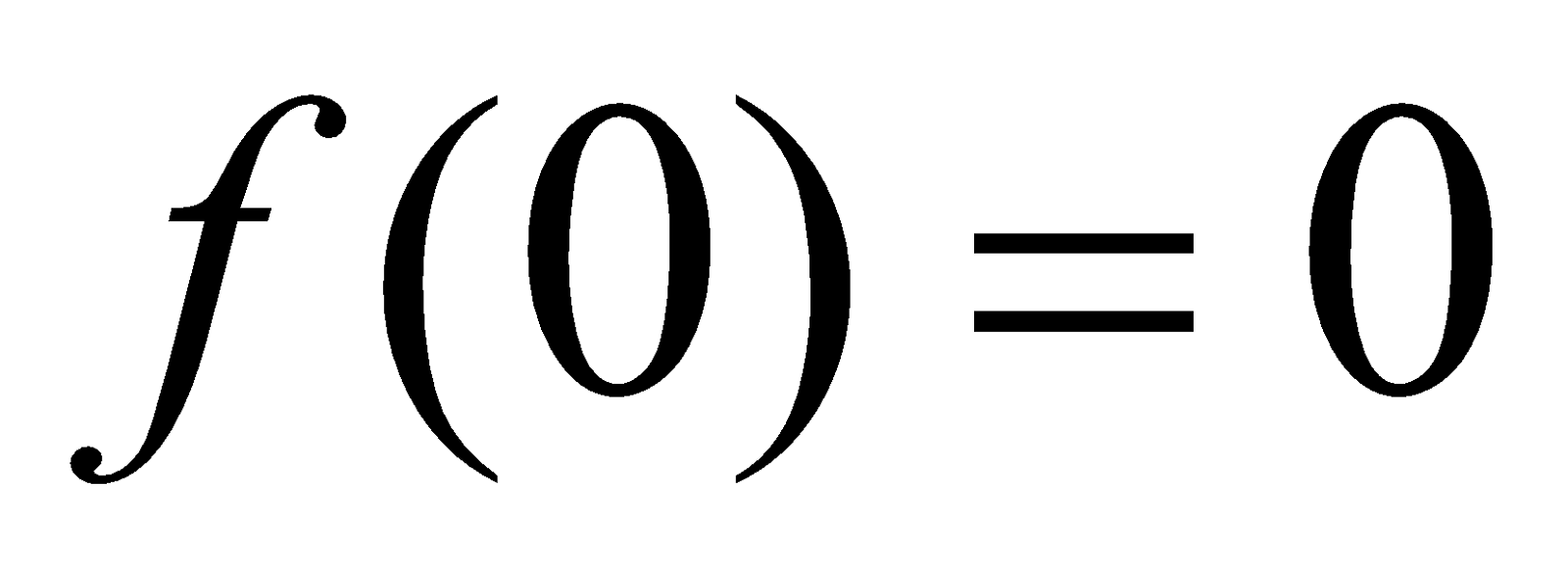. Hence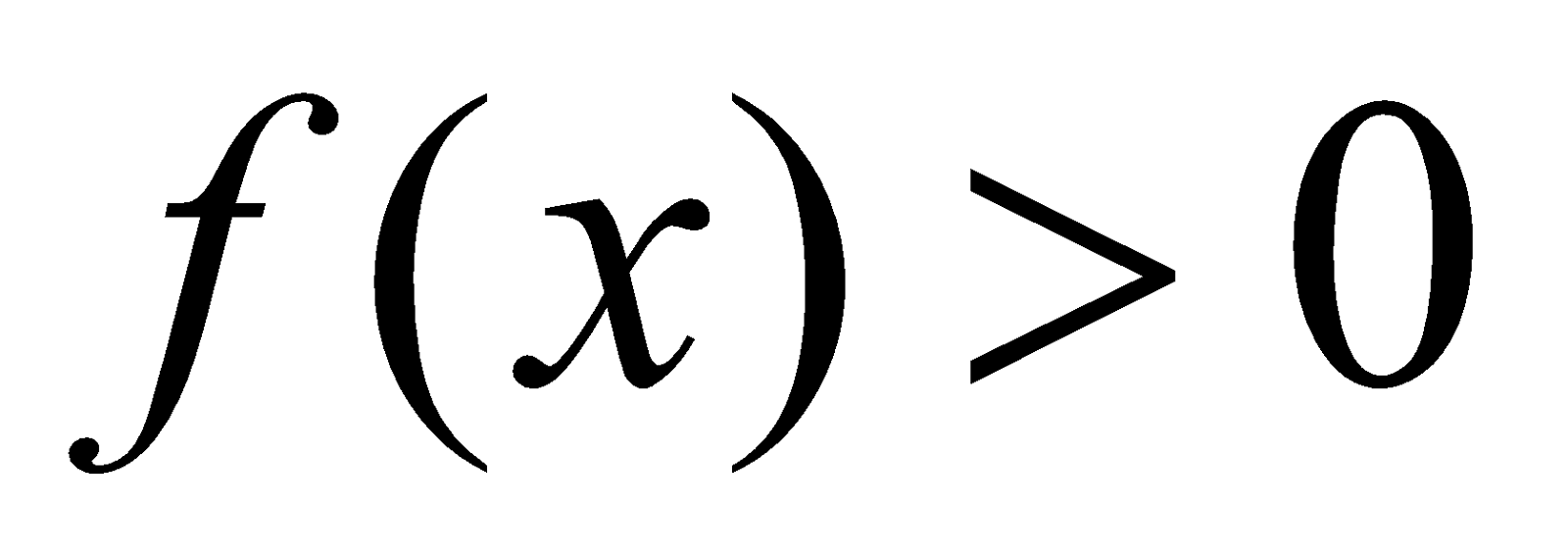at a specified interval. The inequality is proved.

As practice shows, many students, after doing this task, feel the need to generalize the original inequality by introducing into it a parameter. As a result, the hypothesis follows naturally: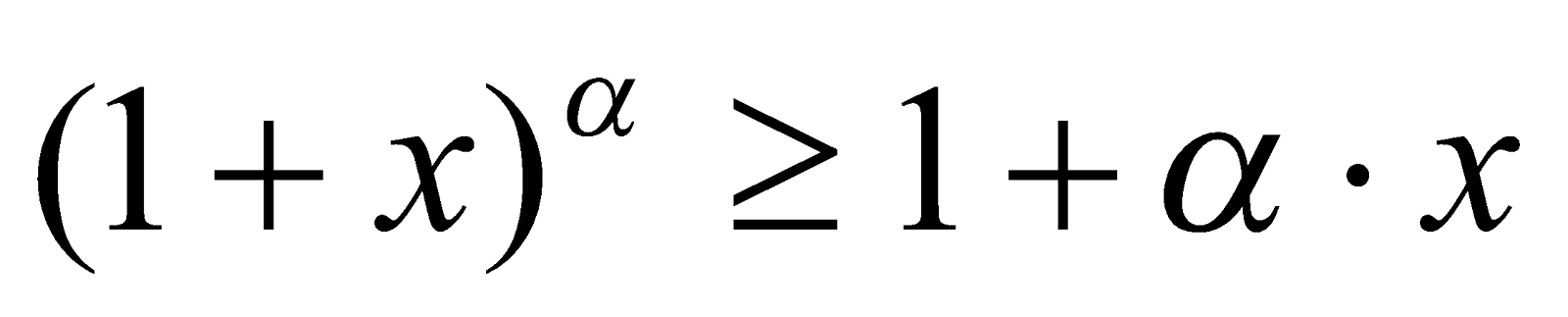if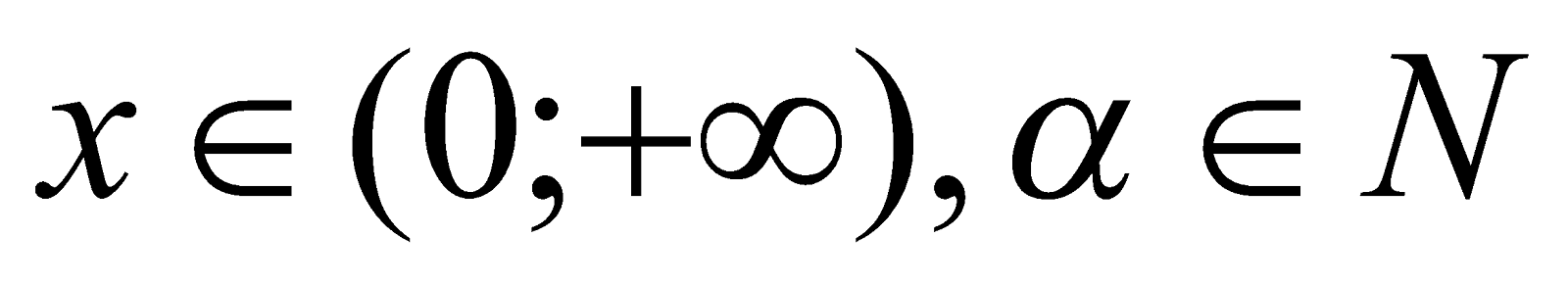. This hypothesis is tested using the same reasoning.

### V.    CONCLUSION

Efficient and purposeful work on the formation of the motivation of learning mathematics is not limited to the periodic use of various methods at different stages of the learning process. Such work should be a constant component of the learning process providing a conscious regulation of students own search activity.

As evidenced by our own teaching experience, the methodological requirements discussed above promote awareness among students of the "openness" of their personal experience. These requirements are realized through the comprehensive interaction between the teacher and the student on the basis by the unity of the motives and purposes of education. It creates prerequisites for the formation of personal responsibility for the results of learning and determines the best perspectives for personal growth.

### REFERENCES

1. Hadamard, J. (1970). The psychology of invention in the mathematical field. Мoscow: Sov. radio.
2. Aleksandrova, P. (1978). Dimension theory and related issues: general articles. Moscow: Science.
3. Bell, E. (1979). Creators mathematics. Moscow: Education.
4. Birkhoff, J. (1977). Mathematics and Psychology. Мoscow: Sov. radio.
5. Weyl, H. (1968) Symmetry. Moscow: Science.
6. Vilyunas, V. (1990). Psychological mechanisms of human motivation. -Moscow: Moscow State University.
7. Cauchy, A. & Newman, J. (1972). Lost and found a paradox. Mathematicians about mathematics (pp.8-24). Moscow: Knowledge.
8. Morris, K. (1980). Mathematics. The Loss of Certainty. Oxford: Oxford University Press.
9. Courant, R. & Robbins, H. (1967). What is mathematics? Moscow: Moscow State University.
10. Lakatos, I. (1967). Proof and refutation. Moscow: Science.
11. Mader, V. (1994). Introduction to the methodology of mathematics. Мoscow: Interpracs.
12. Melnicov, I. & Sergeev, I. (1990). How to solve mathematical problems. Moscow: Moscow State University.
13. Menchinskaya, N. (1989). Problems of teaching and intellectual development of the student. -Moscow: Education.
14. Kulyutkina, Y. & G. Suhobskoy, G. (1990). Teacher thinking: Personal mechanisms and conceptual apparatus. Moscow: Education.
15. Poincaré, A. (1990). About science. -Moscow: Science.
16. Rodionov, M. Shershakova, V., & Nikitina, O. (1997). Collection mathematical problems. Penza: IPK & PRO.
17. Rodionov, M. Shershakova, V., & Marina, E. (2001). From the simple to the complex. Basic methods of solving equations and inequalities in school. Penza: PGPU.
18. Khan, G. (1972). Crisis intuition. Mathe- maticians about mathematics (pp.25-42). Moscow: Knowledge.
19. Heckhausen, H. (1986). Motivation and action. (Vol.1, pp.387) Moscow: Pedagogy.
20. Khinchin, A. (1948). Eight lectures of mathematical analysis. Moscow: OGIZ.
21. Esaulov, A. Psychology of problem solving. (1972). Moscow: High School.
22. Yakimanskaya, C. (2002). Student-oriented education in the modern school. Moscow: September.
23. Yastrebinetsky, G. (1986.) Problems with parameters. Moscow: Education.#### For Authors

/symposiums/webinars, networking opportunities, and privileged benefits.
Authors may submit research manuscript or paper without being an existing member of LJP. Once a non-member author submits a research paper he/she becomes a part of "Provisional Author Membership".

Know more#### For Institutions

Society flourish when two institutions come together." Organizations, research institutes, and universities can join LJP Subscription membership or privileged "Fellow Membership" membership facilitating researchers to publish their work with us, become peer reviewers and join us on Advisory Board.

Know more#### For Subscribers

Subscribe to distinguished STM (scientific, technical, and medical) publisher. Subscription membership is available for individuals universities and institutions (print & online). Subscribers can access journals from our libraries, published in different formats like Printed Hardcopy, Interactive PDFs, EPUBs, eBooks, indexable documents and the author managed dynamic live web page articles, LaTeX, PDFs etc.

Know more

## Introducing DeepReview

##### Artificial Intelligence Based 3rd Peer Reviewer
Meet DeepReview, our 3rd reviewer in the extensive double-blind peer-review process. It's a new generation of Artifical Intelligence that works on deep neural network of machine learning to review research papers. At London Journals Press, we follow an exhaustive process of peer-review, and each article is reviewed by at least two peer reviewers and a team of editorial board members. DeepReview acts as the third peer reviewer that can detect writing styles, plagiarism (for the second time), grammar, contextual spellings, vocabulary, and quality of the article without any human biasing